# JP5441309B2 - Oscillator device and optical deflection device - Google Patents

## Info

Publication number
JP5441309B2
JP5441309B2 JP2007023881A JP2007023881A JP5441309B2 JP 5441309 B2 JP5441309 B2 JP 5441309B2 JP 2007023881 A JP2007023881 A JP 2007023881A JP 2007023881 A JP2007023881 A JP 2007023881A JP 5441309 B2 JP5441309 B2 JP 5441309B2
Authority
JP
Japan
Prior art keywords
time
oscillator
φ
vibration
drive
Prior art date
Legal status (The legal status is an assumption and is not a legal conclusion. Google has not performed a legal analysis and makes no representation as to the accuracy of the status listed.)
Active
Application number
JP2007023881A
Other languages
Japanese (ja)
Other versions
JP2008040460A (en
JP2008040460A5 (en
Inventor

Original Assignee
キヤノン株式会社
Priority date (The priority date is an assumption and is not a legal conclusion. Google has not performed a legal analysis and makes no representation as to the accuracy of the date listed.)
Filing date
Publication date
Priority to JP2006035491 priority Critical
Priority to JP2006035491 priority
Priority to JP2006189947 priority
Priority to JP2006189947 priority
Application filed by キヤノン株式会社 filed Critical キヤノン株式会社
Priority to JP2007023881A priority patent/JP5441309B2/en
Publication of JP2008040460A publication Critical patent/JP2008040460A/en
Publication of JP2008040460A5 publication Critical patent/JP2008040460A5/ja
Application granted granted Critical
Publication of JP5441309B2 publication Critical patent/JP5441309B2/en
Application status is Active legal-status Critical
Anticipated expiration legal-status Critical

## Images

•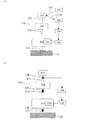•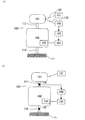•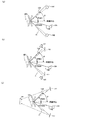•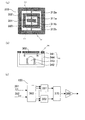•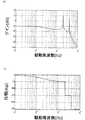•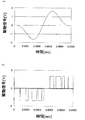•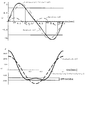••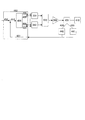•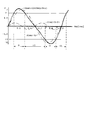•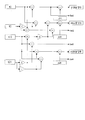•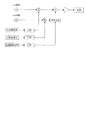•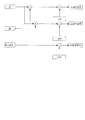•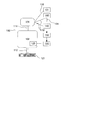•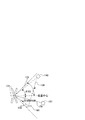•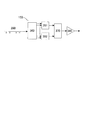•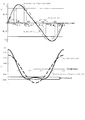•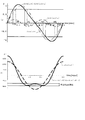••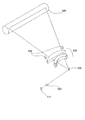•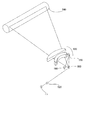•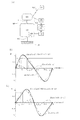•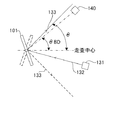•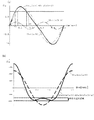•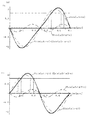•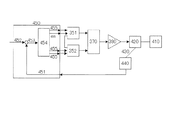•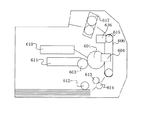•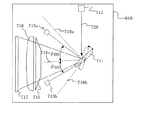•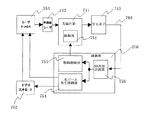•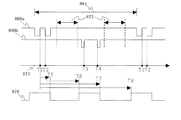•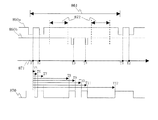•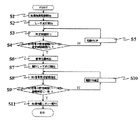•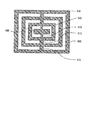•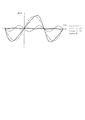•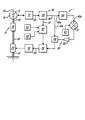## Classifications

• GPHYSICS
• G02OPTICS
• G02BOPTICAL ELEMENTS, SYSTEMS, OR APPARATUS
• G02B26/00Optical devices or arrangements using movable or deformable optical elements for controlling the intensity, colour, phase, polarisation or direction of light, e.g. switching, gating, modulating
• G02B26/08Optical devices or arrangements using movable or deformable optical elements for controlling the intensity, colour, phase, polarisation or direction of light, e.g. switching, gating, modulating for controlling the direction of light
• G02B26/0816Optical devices or arrangements using movable or deformable optical elements for controlling the intensity, colour, phase, polarisation or direction of light, e.g. switching, gating, modulating for controlling the direction of light by means of one or more reflecting elements
• G02B26/0833Optical devices or arrangements using movable or deformable optical elements for controlling the intensity, colour, phase, polarisation or direction of light, e.g. switching, gating, modulating for controlling the direction of light by means of one or more reflecting elements the reflecting element being a micromechanical device, e.g. a MEMS mirror, DMD

## Description

The present invention relates to a technique related to the technical field of an oscillating device having a plurality of oscillating bodies, and more particularly to an apparatus suitable for an optical deflecting device. The present invention also relates to an image forming apparatus such as a scanning display, a laser beam printer, and a digital copying machine using the optical deflection apparatus.

The conventionally proposed resonant optical deflecting device has the following characteristics as compared with an optical scanning optical system using a rotating polygon mirror such as a polygon mirror. That is, it is possible to greatly reduce the size of the optical deflecting device, to reduce power consumption, and to prevent the mirror surface from tilting theoretically.

On the other hand, in the resonance type deflector, since the deflection angle (displacement angle) of the mirror changes sinusoidally in principle, the angular velocity is not constant. In order to correct this characteristic, methods described in Patent Document 1 and Patent Document 2 have been proposed.

In Patent Document 1, triangular wave driving is realized by using a resonant deflector having a vibration mode having a fundamental frequency and a frequency three times that of the fundamental frequency. FIG. 35 shows a micromirror that realizes substantially triangular wave driving. The optical deflection device 12 includes oscillating bodies 14 and 16, torsion springs 18 and 20, drive units 23 and 50, detection units 15 and 32, and a control circuit 30. This micromirror has a resonance frequency that is approximately three times the fundamental resonance frequency, and is driven at a combined frequency of the fundamental frequency and three times the frequency. As a result, the oscillating body 14 having a mirror surface is driven by a triangular wave drive, and the deflection angle of the oscillating body 14 realizes light deflection with less change in angular velocity than the sine drive. At this time, the vibrations of the oscillating body 14 are detected by the detection units 15 and 32, the drive signal necessary for realizing the triangular wave is generated by the control circuit 30, and the micromirrors are driven by the drive units 23 and 50.

Patent Document 2 discloses a micro oscillating body in which a system composed of a plurality of torsion springs and a plurality of movers has a plurality of separated natural vibration modes. In this micro oscillating body, among the separated natural vibration modes, there are a reference vibration mode that is a natural vibration mode of a reference frequency and an even-number vibration mode that is a natural vibration mode of a frequency that is substantially an even multiple of the reference frequency. To do. In Patent Document 2, saw-tooth drive or the like is realized by vibrating the micro oscillating body in these vibration modes.

In Patent Document 3, in order to detect the scanning position of a light beam deflected by a deflection mirror driven by a sine wave, the time when the deflected and scanned light beam passes a predetermined position is detected by an optical sensor. The deflection mirror is controlled using the time.
U.S. Pat. JP 2005-208578 A JP-A-2005-292627

According to the above document, triangular wave driving and sawtooth wave driving of the oscillating body of the deflector is realized, but further improvement is required for the controllability of the deflection angle of the oscillating body. Accordingly, an object of the present invention is to control the deflection angle (displacement angle) of the oscillator of the oscillator device with high accuracy.

In view of the above problems, the oscillator device according to the first aspect of the present invention is configured as follows.
The first oscillator, the second oscillator, the first torsion spring connecting the first oscillator and the second oscillator, and the first torsion spring connected to the second oscillator A vibration system having at least a second torsion spring having a torsion axis common to the torsion axis, and a support portion for supporting the vibration system. The oscillator device according to the present invention further includes a drive unit that drives a vibration system so that at least one of the oscillators has a vibration represented by an expression including a sum of a plurality of time functions, and at least one of the oscillators. A signal output unit that outputs a signal according to the displacement of the oscillator, and a drive control unit that controls the drive unit so that at least one of the amplitude and phase of the time function becomes a predetermined value based on the output signal of the signal output unit With.

The oscillator device according to the second aspect of the present invention is configured as follows.
The first oscillator, the second oscillator, the first torsion spring connecting the first oscillator and the second oscillator, and the first torsion spring connected to the second oscillator A vibration system having at least a second torsion spring having a torsion axis common to the torsion axis, and a support portion for supporting the vibration system. The oscillator device according to the present invention further includes: A 1 sinωt + A 2 , where at least one displacement of the oscillator is A 1 , A 2 is amplitude, φ is a relative phase difference, ω is an angular frequency, and t is time. A drive unit that drives the vibration system so that the vibration is expressed by a mathematical expression (n is an integer of 2 or more) including at least a sin (nωt + φ) term, and a signal according to the displacement of at least one of the rocking bodies. And a drive control unit that controls the drive unit so that at least one of A 1 , A 2 , and φ in the mathematical formula becomes a predetermined value based on the output signal of the signal output unit.

The oscillator device according to the third aspect of the present invention is configured as follows.
The first oscillator, the second oscillator, the first torsion spring connecting the first oscillator and the second oscillator, and the first torsion spring connected to the second oscillator A vibration system having at least a second torsion spring having a torsion axis common to the torsion axis, and a support portion for supporting the vibration system. In the oscillator device of the present invention, when at least one of the oscillators has A 1 and A 2 as amplitude, φ as a relative phase difference, ω as an angular frequency, and t as time, the displacement θ (t) ,
θ (t) = A 1 sin ωt + ΣA n sin (nωt + φ n-1 ) (n is an integer of 2 or more)
Based on a drive unit that drives the vibration system to generate a vibration represented by: a signal output unit that outputs a signal according to the displacement of at least one of the swinging bodies, and an output signal of the signal output unit a 1, a 2 of the formula and an ··· a n, φ 1, φ 2, the drive control unit in which at least one ··· φ n-1 controls the driving unit to a predetermined value .

The oscillator device according to the fourth aspect of the present invention is configured as follows.
Connecting the support part, the first oscillator, the second oscillator, the first torsion spring connecting the first oscillator and the second oscillator, the support part and the second oscillator, and The vibration system includes a second torsion spring having a torsion axis common to the torsion axis of the first torsion spring. Furthermore, in the oscillator device of the present invention, when one of the two oscillators has A 1 and A 2 as amplitude, φ as a relative phase difference, ω as an angular frequency, and t as time, the displacement θ (t) With respect to
θ (t) = A 1 sinωt + A 2 sin (2ωt + φ)
The first and second time information when the driving unit that drives the vibration system so that the vibration is represented by one of the two oscillators takes different first displacement angles, and the second displacement angle And a signal output device that outputs the third and fourth time information when taking, and based on the first to fourth time information, at least one of A 1 , A 2 , φ of the formula is a predetermined value A drive control unit for controlling the drive unit is provided.

In the oscillator device of the present invention, the deflection angle of the oscillator can be controlled with high accuracy.

(First embodiment)
The oscillator device according to the first embodiment will be described. As shown in FIGS. 1A and 1B, the oscillator device of the present embodiment includes a first oscillator 101, a second oscillator 102, a first torsion spring 111, and a second oscillator 102. And a vibration system having at least a second torsion spring 112 and a support portion 121 that supports the vibration system. The first torsion spring connects the first oscillating body and the second oscillating body. The second torsion spring is connected to the second oscillating body so as to have a torsion axis common to the torsion axis of the first torsion spring. The vibration system of the present embodiment only needs to have at least two oscillating bodies and two torsion springs. As shown in FIG. 1, the oscillation system is composed of three or more oscillating bodies and three or more torsion springs. May be.

The driving unit 120 applies a driving force to the vibration system, and the driving control unit 150 adjusts the driving unit 120. The drive unit 120 drives the vibration system so that at least one of the plurality of oscillating bodies has a vibration represented by an expression including the sum of a plurality of time functions. The drive control unit 150 supplies the drive unit 120 with a drive signal that gives such vibration to the vibration system.

When the oscillator device of this embodiment is used as an optical deflecting device, a reflection mirror is formed on at least one oscillator. As the reflection mirror, a light reflection film is formed on the surface of the oscillator. If the surface of the oscillator is sufficiently smooth, it can be used as a reflection mirror without forming a light reflection film. The light deflection apparatus further includes a light source 131 that emits a light beam, and scans the light by irradiating the reflection mirror provided on the oscillator with the light beam 132.

The operating principle of the oscillator device of this embodiment will be described. In general, the equation of free vibration of a vibration system including n oscillators and n torsion springs is given by

Here, I k is the inertia moment of the oscillating body, k k is the spring constant of the torsion spring, θ k is the torsion angle of the oscillating body (k = 1... N). When the eigenvalue of M −1 K of this system is λ k (k = 1 to n), the angular frequency (angular frequency) ω k of the natural vibration mode is given by ω k = √ (λ k ).

Oscillator device of the present invention, in the vibration system with n oscillation modes including n number of oscillator comprises n torsion springs, the fundamental frequency and the frequency of an integral multiple thereof in these omega k n- By configuring so that there is one, various motions can be made to the oscillator. In the present invention, the integer multiple includes substantially integer multiples, and the substantially integer multiple refers to a numerical range of about 0.98n to 1.02n times the fundamental frequency (n is an arbitrary integer).

In particular, the oscillator device of the present embodiment is composed of two oscillators and two torsion springs, and is configured so that there is a fundamental frequency and a frequency that is substantially an even multiple thereof in ω k. In this range, it is possible to realize substantially constant angular velocity driving in which fluctuations in the angular velocity of the oscillator are suppressed.

Further, when n = 3, for example, as shown in FIG. 33, the vibration system having the oscillating bodies (101, 102, 103) and the torsion springs (111, 112, 113) is divided into the frequency ratios of the three vibration modes. Is configured to have a 1: 2: 3 relationship. By simultaneously oscillating the vibration system in vibration modes 1 to 3 of this vibration system, it is possible to drive with a smaller fluctuation in angular velocity than in the case of n = 2. Fig. 34 shows the relationship between the displacement angle of the oscillating body and time when this vibration system is driven with the frequency in each vibration mode set to 1: 2: 3 and the amplitude ratio in each vibration mode set to 24: -6: 1. . The fact that the amplitude ratio is displayed as negative means that the displacement from the origin to the 1/2 cycle is negative as in mode 2 in FIG.

Thus, by increasing the number of vibration modes, the fluctuation of the angular velocity of the rocking body in a predetermined range can be further reduced.

In addition, the oscillator device of the present embodiment is configured by two oscillators and two torsion springs, and the oscillator is configured so that there is a fundamental frequency and approximately three times the frequency in ω k. The moving body can be driven in a substantially triangular wave.

As shown in FIGS. 1 (a) and 1 (b), the vibration of a vibration system composed of n oscillators and n torsion springs will be described. This vibration system is configured to be capable of simultaneously generating a vibration motion that moves at a fundamental frequency and a vibration motion that moves at n-1 frequencies that are substantially an integral multiple of the vibration motion. Therefore, in the first aspect of the present embodiment, at least one of the plurality of oscillators is configured to have a vibration represented by an expression including the sum of a plurality of time functions. Note that the expression including the sum of a plurality of time functions includes an expression including a constant term. For example, the case where the constant term is included is a case where a constant DC bias is applied to the drive unit and the origin of the displacement angle of the oscillator (the position where the displacement angle is 0) is shifted.

In the second mode of the present embodiment, the deflection angle θ of the optical deflector (here, measured with reference to the position of the scanning center as shown in FIG. 3) is as follows. The amplitude and angular frequency of the first vibration motion are A 1 and ω, respectively, and the amplitude and angular frequency of the second vibration motion are A 2 and nω (n is an integer of 2 or more), and the first and second vibrations. Let the relative phase difference of motion be φ. Then, the motion of the oscillating body is a vibration represented by a mathematical formula including at least the term A 1 sinωt + A 2 sin (nωt + φ). In particular, when n = 2, the mathematical expression includes at least the term A 1 sinωt + A 2 sin (2ωt + φ), and it is possible to realize substantially constant angular velocity driving in which fluctuation of the angular velocity of the oscillating body in a predetermined range is suppressed. When n = 3, the equation includes at least the term A 1 sinωt + A 2 sin (3ωt + φ), and the oscillator can be driven in a substantially triangular wave. Also in this case, the formula including at least the term A 1 sinωt + A 2 sin (nωt + φ) includes a formula including a constant term.

In the third aspect of the present embodiment, the amplitude and angular frequency of the first oscillation motion each A 1, and omega, and the amplitude and angular frequency of the vibration motion of the n respective A n, a Enuomega, first If the relative phase difference of the nth vibration motion is φ n−1 , the motion of the oscillator can be expressed by the following equation.
θ (t) = A 1 sinωt + ΣA n sin (nωt + φ n-1 ) Equation 2
Here, n is an integer of 2 or more. The value of n can be increased as long as the number of oscillators constituting the oscillator device can be increased. When actually manufacturing an oscillator device, n is preferably about 3 to 5 at the maximum. The driving unit 120 is configured to be able to apply a driving force to the vibration system by an electromagnetic method, an electrostatic method, a piezoelectric method, or the like. In the case of electromagnetic driving, for example, a permanent magnet may be provided in at least one oscillator, and a coil for applying a magnetic field to the permanent magnet may be disposed near the oscillator, or the permanent magnet and the coil may be reversed. It is good also as arrangement. In the case of electrostatic driving, an electrode is formed on at least one oscillating body, and an electrode that applies an electrostatic force to the electrode is formed in the vicinity of the oscillating body. In the case of piezoelectric driving, a piezoelectric element is provided in a vibration system or a support part to apply driving force.

The drive control unit 150 is configured to generate a drive signal such that the vibration system vibrates in the first to third modes, and applies the drive signal to the drive unit.

The drive signal may be, for example, a drive signal obtained by synthesizing a sine wave (FIG. 6 (a)), or may be a pulsed drive signal (FIG. 6 (b)). In the case of a drive signal obtained by synthesizing a sine wave, a desired drive signal can be obtained by adjusting the amplitude and phase of each sine wave. In the case of driving using a pulse-like signal, a desired drive signal can be generated by changing the number, interval, width, etc. of the pulses with time. In addition, any drive signal may be used as long as it can be driven so that the deflection angle of the optical deflection device becomes a desired deflection angle.

In addition, the oscillator device of the present embodiment includes a signal output device that outputs a signal according to the displacement of at least one oscillator of the plurality of oscillators. In FIG. 1A, the signal output device is a light receiving element 140, and in FIG. 1B, the signal output device is a piezoresistor 170. Such a signal output device is used as a displacement angle measuring device. Hereinafter, the signal output device and the displacement angle measuring device are used in the same meaning.

When detecting the displacement angle of the oscillating body using the piezoresistor 170, for example, when the piezoresistor 170 is provided in a torsion spring and the oscillating body takes a certain displacement angle based on a signal output from the piezoresistor 170 The time of is detected. The piezoresistor 170 is produced, for example, by diffusing phosphorus in p-type single crystal silicon. The piezoresistor 170 outputs a signal according to the torsion angle of the torsion spring. Therefore, when measuring the displacement angle of the oscillating body, the piezoresistors 170 are provided on a plurality of torsion springs, and the displacement angle of the oscillating body is obtained based on the information on the torsion angles of the plurality of torsion springs. Can do.

Further, when the displacement angle of the oscillator is detected using the light receiving element 140, the configuration is as follows.
The first light receiving element is arranged at the scanning light irradiation position when the oscillating body takes the first displacement angle, and the second light receiving element is arranged at the scanning light irradiation position when the oscillating body takes the second displacement angle. Place. The first and second light receiving elements may be different elements or the same element. Further, the scanning light may be directly incident on the light receiving element, or the reflected light may be incident on the light receiving element via at least one reflecting member. In short, it is only necessary that at least one light receiving element is arranged so as to receive and detect scanning light at the first and second scanning angles. The signal output device in the invention according to this embodiment may output a signal when a predetermined displacement angle is intermittently reached on the time axis, or a signal corresponding to the displacement continuously on the time axis. It may be output.

In this specification, the deflection angle of the mirror and the scanning angle of the scanning light deflected and scanned by the mirror have a fixed relationship and can be treated equally, so the deflection angle (displacement angle) and the scanning angle have the same meaning. Use.

For example, as shown in FIG. 3A, the first and second light receiving elements may be arranged at the first and second displacement angles, respectively. Further, as shown in FIG. 3 (b), a reflecting member 160 is provided at the first and second displacement angles, and the light (reflected light) reflected by each reflecting member 160 is the first and second light receiving elements. It is good also as a structure which light-receives by 141,142. Further, as shown in FIG. 15, a light receiving element 140 and a reflecting member 160 may be provided at the first and second displacement angles, respectively. In this case, the scanning light having the first displacement angle is detected by the light receiving element 140, and the scanning light having the second displacement angle is reflected by the reflecting member 160 and received by the light receiving element 140 provided at the first displacement angle. . Further, as shown in FIG. 3C, the reflection member 160 is provided at the first and second displacement angles, and the light (reflected light) reflected by each reflection member 160 is received by one light receiving element 140. It is good also as a structure. In this case, the light receiving element is provided in a region opposite to the light source with respect to the oscillator. In the present embodiment, the scanning light passage time is not limited to the form in which the scanning light passage time is measured at the first and second displacement angles, and the scanning light passage time may be measured at a larger displacement angle. .

In the present invention, the displacement angle includes a displacement angle when the oscillator is stationary, that is, a case where the displacement angle is zero.

In the case of the first aspect, the drive control unit 150 drives based on the output signal of the signal output unit so that at least one of the amplitudes and phases of the plurality of time functions representing the oscillatory motion of the oscillator becomes a predetermined value. The unit 120 is controlled.

Further, in the case of the second mode, the oscillatory motion of the oscillator is expressed by a mathematical formula including at least the term A 1 sinωt + A 2 sin (nωt + φ), and the drive unit is controlled as follows. That is, the drive unit 120 is controlled so that at least one of A 1 , A 2 , and φ in the mathematical formula has a predetermined value.

In the case of the third mode, the oscillatory motion of the oscillating body is expressed by Equation 2, so the drive unit is controlled as follows. That is, the drive control unit 150, A 1, A 2 of the formula based on the output signal of the signal output unit, ··· A n, φ 1, φ 2, at least one ··· φ n-1 is given The drive unit 120 is controlled so that the value becomes.

As described above, the oscillator device according to the present embodiment can control the deflection angle of the oscillator with a simple configuration and high accuracy.

In the invention according to the present embodiment, the drive is adjusted based on the information from the signal output device. And as information from the signal output device, using both information from the signal output device when the displacement angle of the oscillator is positive and information from the signal output device when the displacement angle is negative, It is preferable to control the drive. For example, regarding the displacement angle θ of the oscillator, when using information at four times reflecting the displacement from the signal output device, two of the four times are time information when the displacement angle θ of the oscillator is positive The remaining two are preferably time information when the displacement angle θ is negative. This is because it is effective for adjusting both the clockwise rotation and the counterclockwise rotation of the oscillator.

(Second embodiment)
An oscillator device according to a second embodiment will be described. As shown in FIGS. 2 (a) and 2 (b), the oscillator device of the present embodiment includes a first oscillator 101, a second oscillator 102, a first torsion spring 111, and a second oscillator 102. And a vibration system including the second torsion spring 112 and a support portion 121 that supports the vibration system. The first torsion spring 111 connects the first oscillating body 101 and the second oscillating body 102. The second torsion spring 112 connects the support portion 121 and the second oscillating body 102 so as to have a torsion axis common to the torsion axis of the first torsion spring.

In addition, the time information when the driving unit 120 that applies driving force to the vibration system, the driving control unit 150 that adjusts the driving unit, and one of the two oscillators takes different first and second displacement angles is obtained. And a signal output device for outputting. This signal output device is used as a displacement angle measuring device. In FIG. 2A, the displacement angle measuring device is the light receiving element 140, and in FIG. 2B, the displacement angle measuring device is the piezoresistor 170. The method for detecting the displacement angle of the oscillator using the piezoresistor 170 of the present embodiment and the method for detecting the displacement angle of the oscillator using the light receiving element 140 are the same as those described in the first embodiment. It is.

In addition, a reflection mirror is formed on at least one oscillator. When this oscillator device is used as an optical deflection device, a light source 131 for irradiating a light beam is provided, and a light mirror 132 is applied to the reflection mirror provided on the oscillator to scan the light.

The vibration system has a configuration that can simultaneously generate a first vibration motion that moves at a first frequency that is the fundamental frequency and a second vibration motion that moves at a second frequency that is an integer multiple of the fundamental frequency. It has become.

That is, the deflection angle θ (measured here with reference to the position of the scanning center as shown in FIG. 3) of the optical deflecting device of this embodiment is as follows. The amplitude, angular frequency, and phase of the first vibration motion are A 1 , ω 1 , φ 1 , respectively, and the amplitude, angular frequency, and phase of the second vibration motion are A 2 , ω 2 , φ 2 , respectively, and an appropriate time If the time from the origin or the reference time is t, it can be expressed as
θ (t) = A 1 sin (ω 1 t + φ 1 ) + A 2 sin (ω 2 t + φ 2 ) Equation 3-1

In addition, the deflection angle θ of the optical deflector includes the first vibration motion amplitude and angular frequency A 1 and ω 1 , respectively, the second vibration motion amplitude and angular frequency A 2 and ω 2 , and two frequencies, respectively. When the relative phase difference is φ and the time when the appropriate time is the reference time is t, it can be expressed as the following equation.
θ (t) = A 1 sin (ω 1 t) + A 2 sin (ω 2 t + φ) Equation 3-2
Or θ (t) = A 1 sin (ω 1 t + φ) + A 2 sin (ω 2 t) Equation 3-3

Equation 3-3 corresponds to the case where the phase on the fundamental wave ω 1 side may be adjusted during control. Equations 3-1 and 3-2 and 3-3 differ only in the form of expression depending on how to set the reference or origin time, and are points that contain four unknown values (for example, The φ in 3-2 and Formula 3-3 is essentially the same in φ 1 −φ 2 or φ 2 −φ 1 ).

The driving unit 120 can apply a driving force to the vibration system by an electromagnetic method, an electrostatic method, a piezoelectric method, or the like, and has the same configuration as that of the first embodiment.

The drive control unit 150 is configured to generate a drive signal such that the vibration system vibrates at a fundamental frequency and an integral multiple of the fundamental frequency, and applies the drive signal to the drive unit.

The drive signal may be, for example, a drive signal obtained by synthesizing a sine wave (FIG. 6 (a)), or may be a pulsed drive signal (FIG. 6 (b)). In the case of a drive signal obtained by synthesizing a sine wave, a desired drive signal can be obtained by adjusting the amplitude and phase of each sine wave. In the case of driving using a pulse-like signal, a desired drive signal can be generated by changing the number, interval, width, etc. of the pulses with time. In addition, any drive signal may be used as long as it can be driven so that the deflection angle of the optical deflection device becomes a desired deflection angle.

The displacement angle measuring instrument has two different times when the oscillator takes the first displacement angle and two different times when the second displacement angle is taken within one cycle of the first vibration motion. Measure four times.

The drive control unit 150 generates a drive signal obtained by synthesizing the first signal having the first frequency and the second signal having the second frequency and supplies the drive signal to the drive unit 120, and the measured 4 The drive signal is adjusted so that one time becomes a preset time. Then, by supplying the adjusted drive signal to the drive unit 120, the oscillator device can be controlled with high accuracy.

Further, the drive control unit 150 determines the amplitude and phase of the first vibration motion in Equation 3-1 and the amplitude and phase of the second vibration motion in Formula 3-1 from the four times (A 1 , φ 1 , At least one value of A 2 , φ 2 ) can be calculated. Then, the drive control unit 150 can adjust the drive signal so that at least one of these values becomes a preset value.

When adjusting the drive signal, the amplitude component and phase component of the first vibration motion and the amplitude component and phase component of the second vibration motion of the drive signal are adjusted. Here, for example, the amplitude component of the first vibration motion of the drive signal is a component that can change the amplitude of the first vibration motion of the oscillator in the drive signal. The same applies to the other components.

By supplying the drive signal adjusted in this way to the drive unit 120, the oscillator device can be controlled with high accuracy.

In this embodiment, the scanning light passing time is measured at the first and second displacement angles. However, the present invention is not limited to this, and the scanning light is scanned at a larger displacement angle. You may measure the passage time of.

(Third embodiment)
An oscillator device according to a third embodiment will be described. FIG. 2 (a) shows a block diagram of an optical deflecting device using the oscillator device of the present embodiment. The basic configuration is the same as that of the oscillator device of the first and second embodiments. In the present embodiment, as shown in FIG. 3A, in order to detect the scanning light 133, the first and second light receiving elements are arranged at the first and second displacement angles, respectively.

Also in this embodiment, the deflection angle θ of the optical deflecting device has the amplitude, angular frequency, and phase of the first vibration motion as A 1 , ω 1 , φ 1 , and the amplitude, angular frequency, and phase of the second vibration motion, respectively. When A 2 , ω 2 , φ 2 and time are t, respectively, they can be expressed as in the above equation 3-1.

Also, the deflection angle θ is the first vibration motion amplitude and angular frequency A 1 and ω 1 , respectively, the second vibration motion amplitude and angular frequency A 2 and ω 2 , and the relative phase difference between the two frequencies. Can be expressed as Equation 3-2 and Equation 3-3, where φ is t and time is t when an appropriate time is used as a reference.

Here, the desired four different times are detected within one cycle of the first vibration motion by the first and second light receiving elements arranged at the first and second displacement angles. Then, the drive control unit 150 adjusts the drive signal so that the scanning light passes through the first and second light receiving elements at a preset time.

That is, the drive control unit 150 determines the amplitude and phase of the first vibration motion and the amplitude and phase of the second vibration motion in Formula 3-1 from the four times (A 1 , φ 1 , A in Formula 3-1 2 , φ 2 ) can be calculated. As a result, the desired deflection angle θ of the optical deflecting device can be obtained. At this time, the four times are as follows when the deflection angles corresponding to the positions of the first and second light receiving elements are θ BD1 and θ BD2 (see FIG. 3A), respectively.
At a certain time t 1 and t 2 θ (t 1 ) = θ (t 2 ) = θ BD1 Formula 4
At a certain time t 3 and t 4 θ (t 3 ) = θ (t 4 ) = θ BD2 formula 5

That is, the drive control unit 150 can uniquely determine the amplitude and phase of the first and second vibration motions by setting the four times to arbitrary desired times. More specifically, the drive control unit 150 generates a drive signal and applies the drive signal to the drive unit 120 in order to set the four times to the preset time, and thereby each of the first and second vibration motions. Adjust amplitude, phase or relative phase difference.

The drive signal may be, for example, a drive signal obtained by synthesizing a sine wave (FIG. 6 (a)), or may be a pulsed drive signal (FIG. 6 (b)). In the case of a drive signal obtained by synthesizing a sine wave, a desired drive signal can be obtained by adjusting the amplitude and phase of each sine wave. In the case of driving using a pulse-like signal, a desired drive signal can be generated by changing the number, interval, width, etc. of the pulses with time. In addition, any drive signal may be used as long as it can be driven so that the deflection angle of the optical deflection device becomes a desired deflection angle.

(Fourth embodiment)
An oscillator device according to a fourth embodiment will be described. FIG. 14 shows a block diagram of an optical deflecting device using the oscillator device of the present embodiment. The basic configuration is the same as that of the oscillator device of the first and second embodiments. In the present embodiment, in the reciprocal scanning of each cycle, the scanning light 133 directly passes through the light receiving element 140 twice and is deflected twice by the reflecting plate 160. The deflected light 134 deflected by the reflecting plate 160 passes through the same light receiving element 140 twice. The drive control unit 150 generates a drive signal to the drive unit 120 at four times when the scanning light 133 passes through the light receiving element 140.

FIG. 15 shows the deflection angle θ of the present optical deflection apparatus. The oscillator 101 has a reflection mirror on the surface, and scans the light beam 132 from the light source 131. The light deflecting device has a light receiving element and a reflecting plate, and the light receiving element 140 and the reflecting plate 160 are arranged at positions of deflection angles smaller than the maximum deflection angle of the light deflecting device. In FIG. 15, the light receiving element 140 and the reflecting plate 160 are arranged in the direct optical path of the scanning light of the optical deflecting device. However, as described above, the light receiving element 140 and the reflecting light path 140 are further deflected by another reflecting plate or the like. A reflector 160 may be disposed.

Also in this embodiment, the deflection angle θ of the optical deflecting device has the amplitude, angular frequency, and phase of the first vibration motion as A 1 , ω 1 , φ 1 , and the amplitude, angular frequency, and phase of the second vibration motion, respectively. When A 2 , ω 2 , φ 2 and time are t, respectively, they can be expressed as in the above equation 3-1.

Also, the deflection angle θ is the first vibration motion amplitude and angular frequency A 1 and ω 1 , respectively, the second vibration motion amplitude and angular frequency A 2 and ω 2 , and the relative phase difference between the two frequencies. Can be expressed as Equation 3-2 and Equation 3-3, where φ is t and time is t when an appropriate time is used as a reference.

Here, the light receiving element and the reflecting plate are arranged at a desired position where the scanning light is irradiated, and desired four different times are detected within one cycle of the first vibration motion. Then, the drive control unit 150 adjusts the drive signal so that the scanning light passes on the light receiving element and the reflection plate at a preset time.

That is, the drive control unit determines the amplitude and phase of the first vibration motion and the amplitude and phase of the second vibration motion in Equation 3-1 from the four times (A 1 , φ 1 , A 2 in Equation 3-1 , Φ 2 ) can be calculated. As a result, the desired deflection angle θ of the optical deflecting device can be obtained. At this time, the four times are as follows when the deflection angles corresponding to the positions of the light receiving element and the reflecting plate are θ BD and θ MIRROR (see FIG. 15), respectively.
At some time t 1 and t 2 , θ (t 1 ) = θ (t 2 ) = θ BD formula 6
At some time t 3 and t 4 θ (t 3 ) = θ (t 4 ) = θ MIRROR Equation 7

That is, the drive control unit 150 makes the four passage times (t 1 , t 2 , t 3 , t 4 ) arbitrary desired times, thereby uniquely setting the amplitude and phase of the first and second vibration motions. Can be determined. More specifically, the drive control unit 150 generates a drive signal and applies the drive signal to the drive unit 120 in order to set the four times to the preset time, and thereby each of the first and second vibration motions. Adjust amplitude, phase or relative phase difference.

The drive signal may be, for example, a drive signal obtained by synthesizing a sine wave (FIG. 6 (a)), or may be a pulsed drive signal (FIG. 6 (b)). In the case of a drive signal obtained by synthesizing a sine wave, a desired drive signal can be obtained by adjusting the amplitude and phase of each sine wave. In the case of driving using a pulse-like signal, a desired drive signal can be generated by changing the number, interval, width, etc. of the pulses with time. In addition, any drive signal may be used as long as it can be driven so that the deflection angle of the optical deflection device becomes a desired deflection angle.

(Fifth embodiment)
An oscillating device according to a fifth embodiment will be described. FIG. 22 shows a block diagram of an optical deflecting device using the oscillator device of the present embodiment. The basic configuration is the same as that of the oscillator device of the first and second embodiments. The differences are as follows. As shown in FIG. 22A, which is a block diagram showing the optical deflecting device according to the present embodiment, the drive control unit 150 includes a vibration mode conversion unit 151. The drive control unit 150 generates a first drive signal obtained by synthesizing a first signal having a fundamental frequency and a second signal having a second frequency that is an integer multiple of the fundamental frequency. Further, the drive control unit 150 having the vibration mode conversion unit 151 adds a phase to at least one of the first and second signals, and generates a second drive signal by combining these signals, thereby generating the first drive The signal and the second drive signal can be selectively supplied. As an example, FIG. 22B shows the deflection angle θ at the time of driving the first vibration mode of the vibration system before applying the desired phase, and the second vibration mode of the vibration system after adding the desired phase. The deflection angle θ at the time of driving is shown in FIG. In the illustrated example, the first vibration motion is represented by A 1 sin (ω 1 t), the second vibration motion is represented by A 2 sin (ω 2 t + φ), and the second vibration mode is driven when the second vibration mode is driven. The phase of π is added only to the motion to obtain A 2 sin (ω 2 t + φ + π). As indicated by the solid line curves in FIGS. 22B and 22C, the scanning light 133 passes through the light receiving element 140 two times for a total of four times. The drive control unit 150 calculates a drive signal for making the first vibration motion and the second vibration motion a desired motion from the four passage times. Based on the calculated drive signal, the drive unit 120 controls the vibration system 100 to perform a desired vibration motion.

FIG. 23 shows the deflection angle of the optical deflecting device of this embodiment. The oscillator 101 has a reflection mirror on the surface, and scans the light beam 132 from the light source 131. The light deflection apparatus has one light receiving element 140, and the light receiving element 140 is disposed at a position of a deflection angle smaller than the maximum deflection angle of the light deflection apparatus. In FIG. 23, the light receiving element 140 is arranged in the optical path of the optical deflecting device. However, the light receiving element 140 may be arranged in the optical path of the scanning light further deflected by another reflecting plate or the like.

The amplitude, angular frequency, and phase of the first vibration motion are A 1 , ω 1 , and φ 1 , respectively, and the amplitude, angular frequency, and phase of the second vibration motion are A 2 , ω 2 , φ 2 , and the time is t, respectively. deflection angle theta a of the optical deflecting device in the first oscillation mode with can be expressed by the following equation.
θ a (t) = A 1 sin (ω 1 t + φ 1 ) + A 2 sin (ω 2 t + φ 2 ) Equation 8

Further, the deflection angle θ b of the second vibration mode optical deflection apparatus when the desired phases φ 1 ′ and φ 2 ′ are added to the phases φ 1 and φ 2 by the vibration mode converter 151 is expressed by the following equation: Can express.
θ b (t) = A 1 sin (ω 1 t + φ 1 + φ 1 ′) + A 2 sin (ω 2 t + φ 2 + φ 2 ′) Equation 9

The light receiving element 140 is arranged at a desired position where the scanning light is irradiated, and desired four different times are detected with a certain point in one cycle of the first vibration motion as the origin. Then, the drive control unit 150 adjusts the drive signal so that the scanning light passes through the light receiving element at a preset time. That is, the desired deflection angle θ of the optical deflector can be obtained by calculating the amplitude, angular frequency, and phase of the first and second vibration motions from the four times and adjusting the drive signal.

The four times are as follows at certain times t 1 and t 2 and certain times t 3 and t 4 when the deflection angle corresponding to the position of the light receiving element 140 is θ aBD .
θ a (t 1 ) = θ a (t 2 ) = θ aBD equation 10
θ b (t 3 ) = θ b (t 4 ) = θ aBD equation 11

The drive control unit 150 can uniquely determine the amplitude and phase of the first and second vibration motions by setting the four passage times (t 1 , t 2 , t 3 , t 4 ) to desired set times, respectively. . More specifically, the drive control unit 150 generates a drive signal and applies the drive signal to the drive unit 120 in order to set the four times to preset times, so that each of the first and second vibration motions is performed. Are adjusted in amplitude and phase A 1 , φ 1 , A 2 , φ 2 .

Or, the amplitude and angular frequency of the first vibration motion are A 1 and ω 1 , the amplitude and angular frequency of the second vibration motion are A 2 and ω 2 , the relative phase difference between the two frequencies is φ, and the appropriate time is 0 and the time when when a t, the deflection angle theta a of the optical deflecting device in the first oscillation mode can be expressed by the following equation.
θ a (t) = A 1 sin (ω 1 t) + A 2 sin (ω 2 t + φ) Equation 12

In addition, when the desired phases φ 1 ′ and φ 2 ′ are added to the first and second vibration motions by the vibration mode conversion unit 151 , the deflection angle θ b of the second vibration mode of the optical deflector is expressed by the following equation: Can be expressed as
θ b (t) = A 1 sin (ω 1 t + φ 1 ′) + A 2 sin (ω 2 t + φ + φ 2 ′) Equation 13

Also here, the light receiving element 140 is arranged at a desired position where the scanning light is irradiated, and desired four different times are detected with a certain point in one cycle of the first vibration motion as the origin. Then, the drive control unit 150 adjusts the drive signal so that the scanning light passes through the light receiving element at a preset time.

That is, the desired deflection angle θ of the optical deflector can be obtained by calculating the amplitude, angular frequency, and phase of the first and second vibration motions from the four times and adjusting the drive signal.

The four times are as follows at certain times t 1 and t 2 and certain times t 3 and t 4 when the deflection angle corresponding to the position of the light receiving element 140 is θ aBD .
θ a (t 1 ) = θ a (t 2 ) = θ aBD Equation 14
θ b (t 3 ) = θ b (t 4 ) = θ aBD Equation 15

The drive control unit 150 can uniquely determine the amplitude and phase of the first and second vibration motions by setting the four passage times (t 1 , t 2 , t 3 , t 4 ) to desired set times, respectively. . More specifically, the drive control unit 150 generates a drive signal and applies the drive signal to the drive unit 120 in order to set the four times to a preset time, whereby the first vibration motion amplitude A 1 , The amplitude A 2 and the phase difference φ of the second vibration motion are adjusted.

Also in the present embodiment, the drive signal may be, for example, a drive signal obtained by synthesizing a sine wave (FIG. 6 (a)) or a pulsed drive signal (FIG. 6 (b)). In the case of a drive signal obtained by synthesizing a sine wave, a desired drive signal can be obtained by adjusting the amplitude and phase of each sine wave. In the case of driving using a pulse-like signal, a desired drive signal can be generated by changing the number, interval, width, etc. of the pulses with time. In addition, any drive signal may be used as long as it can be driven so that the deflection angle of the optical deflection device becomes a desired deflection angle.

Hereinafter, more specific embodiments of the present invention will be described with reference to the drawings.

(Example 1)
An optical deflecting device according to Embodiment 1 of the present invention will be described. The block diagram of the optical deflecting device of Embodiment 1 is the same as that shown in FIG. FIG. 4 is a specific configuration diagram of the present embodiment. FIG. 4A is a top view of the vibration system of the present optical deflection apparatus. The plate member 300 is produced by etching a silicon wafer. The flat rocking body 301 is supported by two torsion springs 311a and 311b at the top and bottom in the drawing. A light reflection film (reflection mirror) 331 is formed on the upper surface of the oscillator 301. The frame-shaped oscillating body 302 supports torsion springs 311a and 311b on the inside thereof, and is supported by two torsion springs 312a and 312b in the upper and lower directions in the figure. The frame-shaped support frame 321 supports the torsion springs 312a and 312b on the inside thereof.

In this embodiment, the oscillating body 301 and the oscillating body 302 are fixed to each other by two torsion springs, but each oscillating body may be supported only on one side by one torsion spring. For example, the oscillating body 301 may be fixed by one torsion spring 311b, and the oscillating body 302 may be fixed by two torsion springs 312a and 312b. Conversely, the oscillating body 301 may be fixed by two torsion springs 311a and 311b, and the oscillating body 302 may be fixed by one torsion spring 312b.

The vibration system including the oscillating bodies 301 and 302 and the torsion springs 311 and 312 has two vibration modes, but is adjusted so that one of their frequencies is approximately twice the other. As an example, the inertia of the oscillator 301 and 302 mode - the placement and I 1, I 2, torsion springs 311a, k 1 a spring constant consisting 311b, when the torsion spring 312a, the spring constant composed of 312b and k 2, 2 One natural angular frequency is uniquely determined. In this embodiment, ω 1 = 2π × 2000 [ rad / s], ω 2 = 2π × 4000 inertia so that [rad / s] moments I 1, I 2, and the spring constant k 1, k 2 It is adjusted.

FIG. 4B is a schematic diagram for explaining a drive unit of the present optical deflection apparatus. In the drawing, the plate member 300 shows a cross section cut along a cutting line 390 of FIG. A permanent magnet 341 is bonded to the lower surface of the oscillator 302, and the plate member 300 is bonded to a yoke 344 made of a material having high magnetic permeability. A core 343 made of a material having high magnetic permeability is disposed at a portion of the yoke 344 opposite to the permanent magnet 341, and a coil 342 is wound around the core 343. The permanent magnet 341, the coil 342, the core 343, and the yoke 344 constitute an electromagnetic actuator (drive unit) 340. When a current is passed through the coil 342, torque acts on the permanent magnet 341, and the oscillator 302 Driven.

5 (a) and 5 (b) show the transmission characteristics of the displacement angle of the oscillator 301 when a voltage is applied to the coil. FIG. 5 (a) shows the relationship between gain (displacement angle / applied voltage) and drive frequency, and FIG. 5 (b) shows the relationship between applied voltage and phase difference between displacement angle and drive frequency. Figure 5 gain of the oscillation mode of the omega 2 to omega 1 vibration mode as shown in (a) (efficiency) is different, the vibration mode of FIG. 5 with respect to omega 1 vibration mode as shown in (b) omega 2 Is 180deg behind in phase.

FIG. 4C shows the control unit 150 of the present light deflection apparatus. In the figure, reference numerals 351 and 352 denote arbitrary waveform generators that generate sine waves of 2000 Hz and 4000 Hz. The phase and amplitude of each sine wave can be arbitrarily changed by a command from the calculation unit 360. The two generated sine waves are added by an adder 370 and then amplified by an amplifier 380, and a voltage is applied to the coil 342 so that a current flows. The first and second light receiving elements 141 and 142 are arranged as shown in FIG. 3A, and outputs 391 and 392 from the first and second light receiving elements are taken into the calculation unit 360. The calculation unit 360 passes through the first and second light receiving elements 141 and 142 so that the outputs 391 and 392 of the first and second light receiving elements indicate arbitrary values, that is, the scanning light 133 is at an arbitrary time desired. In this manner, the phase and amplitude of each sine wave of the arbitrary waveform generators 351 and 352 are adjusted.

Drive signal obtained by synthesizing two frequencies using arbitrary-wave producing circuits 351 and 352 and the adder 370 in the present embodiment has generated the (FIG. 6 (a)), the command of the arithmetic unit 360 natural angular frequency omega 1 of The voltage waveform within one period may be divided and configured by a large number of pulse trains (FIG. 6 (b)). That is, a PWM (Pulse Width Modulation) driving unit that changes the number of pulses, the interval, the width, etc. with time and changes the amplitude components and phases of the natural angular frequencies ω 1 and ω 2 may be used.

By using the optical deflecting device of this embodiment, it is possible to realize desired arbitrary optical scanning having two frequency components (for example, scanning light whose scanning angle changes in a substantially sawtooth shape).

(Example 2)
An optical deflecting device according to Embodiment 2 of the present invention will be described. The block diagram of the optical deflecting device of this embodiment is the same as that shown in FIG. The configuration is the same as that shown in FIG.

The deflection angle θ of the present optical deflection apparatus is expressed as follows. The amplitude and angular frequency of the first vibration motion are A 1 and ω 1 , the amplitude and angular frequency of the second vibration motion are A 2 and ω 2 , respectively, and the phases of the two frequencies are φ 1 and φ 2 , respectively. To do. Then, assuming that an arbitrary time within one cycle of the first vibration motion is the origin or reference, t is θ (t) = A 1 sin (ω 1 t + φ 1 ) + A 2 in the above equation 3-1. It can be expressed as sin (ω 2 t + φ 2 ).

Here, when A 1 = 1, A 2 = 0.2, φ 1 = 0, φ 2 = 0, ω 1 = 2π × 2000, and ω 2 = 2π × 4000, the deflection angle θ and the angular velocity θ of the present optical deflector The change with time is as shown in Fig.7. The deflection angle θ shown by the solid line in FIG. 7 (a) is closer to a sawtooth wave than the sine wave (shown by a broken line), and the angular velocity θ ′ shown by the solid line in FIG. As compared with the above, the amount of change is smaller in the substantially constant angular velocity region. Note that the unit of the vertical axis in FIG.

In this embodiment, A 1 = 1, A 2 = 0.2, φ 1 = 0, φ 2 = 0, ω 1 = 2π × 2000, and ω 2 = 2π × 4000, but the change amount of the angular velocity θ ′ is a sine wave. Any of A 1 , A 2 , φ 1 , φ 2 , ω 1 , and ω 2 , which is smaller in the substantially equal angular velocity region than that in FIG. Preferably, in a continuous time of 20% or more within one period of the first frequency, the maximum value and the minimum value of the angular velocity θ ′ of the reflection mirror within the time are (maximum value−minimum value) / (maximum). Value + minimum value) <0.1. This is a preferable rough condition required for the optical deflection apparatus, and the same applies to other embodiments.

At this time, 80% of the first and second light receiving elements 141 and 142 A 1, that is respectively arranged from the scanning center of the optical deflecting device deflecting angle θ is 0.8 (the maximum deflection angle as 1) positioned symmetrically Then, it becomes as follows. That is, desired target times t 10 , t 20 , t 30 , and t 40 (see FIG. 7A) for the scanning light 133 to pass through the first and second light receiving elements 141 and 142 are 0.052 msec, 0.154 msec, 0.346msec and 0.448msec. These target times are obtained and stored in advance (this is the same in the following embodiments). Therefore, the times t 1 , t 2 , t 3 , and t 4 when the scanning light 133 passes through the first and second light receiving elements 141 and 142 are set to the desired values as shown in FIGS. By adjusting the drive signal as shown in b) by the control unit 150, the deflection angle θ indicated by the solid line in FIG. 7 can be obtained.

In the present embodiment, the first and second light receiving elements 141 and 142 are arranged at symmetrical positions from the scanning center of the optical deflecting device where the deflection angle θ is 0.8, but may be at an arbitrary deflection angle θ. Preferably, in order to avoid optical interference in a substantially constant velocity region, it is desirable to dispose the first and second light receiving elements 141 and 142 in a range where the absolute value of the deflection angle θ is 0.6 or more and less than 1.0, respectively. . Here, the range where the absolute value of the deflection angle θ is 0.6 or more and less than 1.0 is the range where the deflection angle θ is less than +1.0 and +0.6 or more, and the range is −0.6 or less and more than −1.0. It is. Note that the deflection center of the reflection mirror is zero, and the desired maximum deflection angle is ± 1. This arrangement is the same in the other embodiments.

A more specific method of controlling the deflection angle in this embodiment will be described below. FIG. 8 shows the flow of control.

<A 1 Seigyo_>
First, the control of A 1. In order to perform optical scanning only with the first vibration motion that moves at the fundamental frequency, the frequency of the arbitrary waveform generator 351 is set to an angular frequency of 2000 Hz, and the frequency of the arbitrary waveform generator 352 is set to an arbitrary value including zero other than 2000 Hz and 4000 Hz. Angular frequency. Then, the second vibration motion does not cause resonance vibration, and the deflection angle θ of the optical deflector can be expressed by the following equation.
θ (t) = A 1 sin (ω 1 t) Equation 16

Then, certain times t 1 , t 2 , t 3 and t 4 are set as follows.
θ (t 1 ) = θ (t 2 ) = θ BD1 Formula 17
θ (t 3 ) = θ (t 4 ) = θ BD2 formula 18
Then, at least one of t 2 −t 1 and t 4 −t 3 is arbitrarily set to be 0.102 msec (this is obtained in advance from the change in the target deflection angle θ shown in FIG. 7). By adjusting the amplitude of the waveform generator 351, A 1 can be made the desired A 1 . Since one unknown value is determined, A 1 can be determined in this way.

This step is a step of determining the amplitude of the first oscillating motion of the reflecting mirror on the oscillating body, stopping the second oscillating motion and scanning the light only by the first oscillating motion. This is a step of adjusting as follows, with a certain time within one period of one frequency being zero or a reference. That is, at least one of two different sets of passage times through which the scanning light passes through the first light receiving element and two different sets of passage times through which the scanning light passes through the second light receiving element The amplitude of the first vibration motion is adjusted so that the set time becomes the desired target time.

Thereafter, the frequency of the arbitrary waveform generator 352 is returned to 4000 Hz. In this embodiment, since the optical scanning is performed only by the first vibration motion that moves at the fundamental frequency, the frequency of the arbitrary waveform generator 352 is set to an arbitrary frequency including zero other than 2000 Hz and 4000 Hz. That is, when stopping the second vibration motion, the driving force transmitted from the drive unit to the vibration system is stopped, the periodic driving force of the second frequency is stopped, and other than the first frequency and the second frequency A periodic driving force of the third frequency is added. However, at this time, the amplitude A 2 of the arbitrary waveform generator 352 may be set to zero.

<Φ control>
Next, the phase difference φ between the first and second vibration motions is adjusted to zero. At that time, do as follows.
t 2 −t 1 = t 4 −t 3 Equation 19
And,
t 3 −t 2 > t 30 −t 20 Formula 20
The reason why Expression 19 is required is that the first and second light receiving elements 141 and 142 are arranged at symmetrical positions from the scanning center of the optical deflector. When the phase difference between the arbitrary waveform generators 351 and 352 is adjusted in this way, the phase difference between the first and second vibration motions becomes zero. Again, since there is only one unknown value to be determined, φ can be determined by this method. Expression 20 is a condition for avoiding the vibration motion from having an opposite phase.

This step is a step of determining the relative phase difference between the first vibration motion and the second vibration motion of the reflection mirror. Here, the difference between two different passage times when the scanning light passes through the first light receiving element is equal to the difference between two different passage times when the scanning light passes through the second light receiving element. Similarly, the phase of at least one of the first and second vibration motions is adjusted.

<A2 control>
Subsequently, A 2 is controlled. The times when the scanning light 133 passes through the first and second light receiving elements 141 and 142 are defined as t 1 , t 2 , t 3 , and t 4 . The amplitude of the arbitrary waveform generator 352 is adjusted so that at least one of them satisfies t 1 = 0.052 msec, t 2 = 0.154 msec, t 3 = 0.346 msec, and t 4 = 0.448 msec. This makes it possible to the A 2 and the desired A 2. Again, since there is only one unknown value to determine, A 2 can be determined in this way.

This step is a step of determining the amplitude of the second oscillating motion of the reflecting mirror, so that at least one of the times when the scanning light passes through the first and second light receiving elements is set to a desired value. And adjusting the amplitude of the second vibration motion.

<Confirmation of control completion>
Here, if t 1 , t 2 , t 3 , t 4 are within a certain allowable error range, the control is terminated. If different returns to A 1 control performs the control.

With the above operation, a desired deflection angle θ of the optical deflecting device can be realized. In this embodiment, t 1 , t 2 , t 3 , t 4 , t 10 , t 20 , t 30 , and t 40 are handled as time, but a counter value based on a certain clock may be used. In the present embodiment, t 1 , t 2 , t 3 , t 4 , t 10 , t 20 , t 30 , and t 40 are determined values, but values having a certain error range may be used. These are the same in other embodiments.

(Example 3)
An optical deflecting device according to Embodiment 3 of the present invention will be described. The block diagram of the optical deflecting device of this embodiment is the same as that shown in FIG. The configuration is the same as that shown in FIG.

Again, the amplitude and angular frequency of the first oscillatory motion are A 1 and ω 1 , respectively, the amplitude and angular frequency of the second oscillatory motion are A 2 and ω 2 , respectively, and the phase of each of the two frequencies is φ 1 , φ 2 , t is the time when the origin (0) is an arbitrary reference time within one cycle of the first vibration motion. At this time, the deflection angle θ of the present optical deflection apparatus can also be expressed by θ (t) = A 1 sin (ω 1 t + φ 1 ) + A 2 sin (ω 2 t + φ 2 ) in the above equation 3-1.

Here, when A 1 = 1, A 2 = 0.2, φ 1 = 0, φ 2 = 0, ω 1 = 2π × 2000, and ω 2 = 2π × 4000, the deflection angle θ of the present optical deflecting device is as shown in FIG. As shown in In this embodiment, A 1 = 1, A 2 = 0.2, φ 1 = 0, φ 2 = 0, ω 1 = 2π × 2000, and ω 2 = 2π × 4000, but the change amount of the angular velocity θ ′ is a sine wave. Any of A 1 , A 2 , φ 1 , φ 2 , ω 1 , and ω 2 , which is smaller in the substantially equal angular velocity region than that in FIG.

At this time, 80% of the first and second light receiving elements 141 and 142 A 1, that is, when the deflection angle θ is to extend on the different positions of 0.8, and 0 time the deflection angle θ is 0 (scanning center) When it does, it becomes as follows. That is, the target times t 10 , t 20 , t 30 , and t 40 through which the scanning light 133 passes through the first and second light receiving elements 141 and 142 are 0.052 msec, 0.154 msec, 0.346 msec, and 0.448 msec. Therefore, the drive signal is adjusted by the control unit so that the four detection times t 1 , t 2 , t 3 , and t 4 through which the scanning light 133 passes through the first and second light receiving elements 141 and 142 become the above values. Thus, the deflection angle θ of the optical deflection apparatus shown in FIG. 7 can be obtained.

In the present embodiment, the first and second light receiving elements 141 and 142 are arranged at symmetrical positions from the scanning center of the optical deflecting device where the deflection angle θ is 0.8, but any deflection angle θ may be used. In the present embodiment, the time when the deflection angle θ is 0 is set to 0, but an arbitrary time of one cycle of the angular frequency of the first vibration motion may be set as the origin (0).

The control method of the present embodiment will be described in detail. The scanning light 133 scans the first and second light receiving elements 141 and 142 when the control parameter X including any one of A 1 , A 2 , φ 1 , and φ 2 of the present optical deflecting device slightly changes from the target value. Coefficients and matrix M representing changes in detection times t 1 , t 2 , t 3 , and t 4 passing through are obtained in advance. These are expressed as follows:

Therefore, the operation amounts ΔA 1 , ΔA 2 , Δφ 1 , Δφ 2 of the amplitude and phase of the reflecting mirror are represented by four detection times t 1 , t 2 , t 3 , t 4 and four target times t 10 , t 20 , From the time differences Δt 1 , Δt 2 , Δt 3 and Δt 4 with respect to t 30 and t 40 , the following equation is obtained.

The manipulated variables ΔA 1 , ΔA 2 , Δφ 1 , Δφ 2 are calculated from the time differences Δt 1 , Δt 2 , Δt 3 , Δt 4 from the target times t 10 , t 20 , t 30 , t 40 from the above relational expression. Based on the value, the outputs of the arbitrary waveform generators 351 and 352 are changed. By repeating the above control, it converges at the target times t 10 , t 20 , t 30 and t 40 , and a desired deflection angle θ of the optical deflecting device can be obtained.

The above process is shown in the block diagram of FIG. By deflecting light from the light source 410 by the light deflecting device (reflection mirror) 420, the deflected light 430 passes through the first and second light receiving elements 441 and 442. The control unit 450 calculates a time difference 453 by subtracting the detection time 451 detected by the first and second light receiving elements 441 and 442 and the target time 452. Then, as shown in Equation 23, the operation amount 455 is calculated by performing a matrix operation with the arithmetic unit 454 from the time difference 453, and the arbitrary waveform generators 351, 352, the adder 370, and the amplifier 380 are used to drive the optical deflector 420. Generate the input signal.

Also in this embodiment, for example, a drive signal obtained by synthesizing a sine wave as shown in FIG. 6 (a) may be generated, or a pulse-like drive signal as shown in FIG. 6 (b) may be generated. Good. That is, any drive signal may be used as long as the detection time detected by the light receiving element becomes the target time.

The transfer characteristic of the displacement angle of the rocking body 301 shown in FIG. 5 changes depending on the environmental change such as the environmental temperature and the time-dependent change of the vibration characteristic of the rocking body. Accordingly, the control unit 150 updates the drive waveform for each oscillation period of ω 1 of the optical deflector so as to obtain a desired deflection angle θ of the optical deflector. In the present embodiment, the drive waveform is updated every ω 1 vibration cycle, but it may be controlled at a shorter cycle, for example, the time when the signal is input to the light receiving element. Alternatively, it may be controlled with a period longer than the vibration period of ω 1 of the optical deflector.

(Example 4)
An optical deflecting device according to Embodiment 4 of the present invention will be described. The block diagram of the optical deflecting device of this embodiment is the same as that shown in FIG. The configuration is the same as that shown in FIG. The deflection angle θ of the present optical deflecting device is the first vibration motion amplitude and angular frequency of A 1 and ω 1 , respectively, and the second vibration motion amplitude and angular frequency of A 2 and ω 2 , respectively. When each phase difference is φ and time is t, it can be expressed by the above equation 3-2 or 3-3. Φ in Equation 3-2 and Equation 3-3 is φ 1 −φ 2 or φ 2 −φ 1 .

Here, A 1 = 1, A 2 = 0.2, φ = 0, ω 1 = 2π × 2000, and ω 2 = 2π × 4000. In this embodiment, A 1 = 1, A 2 = 0.2, φ = 0 (φ 1 = 0, φ 2 = 0), ω 1 = 2π × 2000, ω 2 = 2π × 4000, but the angular velocity θ ′ Any A 1 , A 2 , φ 1 , φ 2 , ω 1 , ω 2 in which the amount of change is smaller in the substantially equal angular velocity region than the sine wave may be used. The first and second light receiving elements 141 and 142 80% of A 1, that is placed in mutually different positions the deflection angle θ of 0.8, the scanning light 133 passes through the first and second light receiving elements 141 and 142 the t 10 of the target time t 10, t 20, t 30 , t 40 to a reference time. Then, the relative target times t 20 −t 10 , t 30 −t 10 and t 40 −t 10 from the reference time are 0.102 msec, 0.294 msec and 0.396 msec, and the deflection angle θ of the present optical deflector is as shown in FIG. become. Therefore, control is performed so that the three detection relative times t 2 −t 1 , t 3 −t 1 , and t 4 −t 1 during which the scanning light 133 passes through the first and second light receiving elements 141 and 142 become the above values. By adjusting the drive signal by the unit, the deflection angle θ of the optical deflector shown in FIG. 7 can be obtained. φ 1 and φ 2 are expressed by a relational expression of φ = φ 1 −φ 2 or φ = φ 2 −φ 1 , and the above equation 3-1 in FIG. 7 is changed to the above equation 3-2 or the above equation 3 Converted to -3.

Also in this embodiment, the first and second light receiving elements 141 and 142 are arranged at symmetrical positions from the scanning center of the optical deflecting device where the deflection angle θ is 0.8, but any deflection angle θ may be used.

The control method of the present embodiment will be described in detail. Detection relative time when the scanning light 133 passes through the first and second light receiving elements 141 and 142 when the control parameter X including any one of A 1 , A 2 , and φ of the light deflecting device slightly changes from the target value A coefficient representing a change in t 2 −t 1 , t 3 −t 1 , t 4 −t 1 and a matrix M are obtained in advance. These are expressed as follows:

Therefore, the operation amounts ΔA 1 , ΔA 2 , Δφ of the mirror amplitude and phase are represented by three detection relative times t 2 −t 1 , t 3 −t 1 , t 4 −t 1 and three target times t 20 −t. From the time differences Δt 2 , Δt 3 , and Δt 4 with respect to 10 , t 30 −t 10 and t 40 −t 10 , the following equation is obtained.

The manipulated variables ΔA 1 , ΔA 2 , Δφ are calculated from the time differences Δt 2 , Δt 3 , Δt 4 from the target times t 20 −t 10 , t 30 −t 10 , t 40 −t 10 from the above relational expression. Based on the value, the outputs of the arbitrary waveform generators 351 and 352 are changed. By repeating the above control, it converges at the target times t 10 , t 20 , t 30 and t 40 , and a desired deflection angle θ of the optical deflecting device can be obtained.

The above process will be described with reference to the block diagram of FIG. By deflecting light from the light source 410 by the light deflecting device 420, the deflected light 430 passes through the first and second light receiving elements 441 and 442. The control unit 450 calculates a time difference 453 by subtracting the detection time 451 detected by the first and second light receiving elements 441 and 442 and the target time 452. Further, as shown in Equation 26, the operation amount 455 is calculated by performing a matrix operation with the calculator 454 from the time difference 453, and the arbitrary waveform generators 351 and 352, the adder 370, and the amplifier 380 are used to drive the optical deflector 420. Generate the input signal. In this case, since a reference time t 10, the control amount 455 for the arbitrary-waveform generator 351 shown in FIG. 9 instead of two, or become one, or the control amount 455 for the arbitrary-waveform generator 352 It will be one instead of two. That is, the phase difference φ between the two frequencies can be adjusted by either the arbitrary waveform generator 351 or the arbitrary waveform generator 352.

Also in this embodiment, for example, a drive signal obtained by synthesizing a sine wave as shown in FIG. 6 (a) may be generated, or a pulse-like drive signal as shown in FIG. 6 (b) may be generated. Good. That is, any drive signal may be used as long as the detection time detected by the light receiving element becomes the target time.

With the above control, a desired deflection angle θ of the optical deflecting device can be realized. In this embodiment, t 20 −t 10 , t 30 −t 10 , and t 40 −t 10 are determined values, but may be values having an error range.

(Example 5)
An optical deflecting device according to Embodiment 5 of the present invention will be described. The block diagram of the optical deflecting device of this embodiment is the same as that shown in FIG. The configuration is the same as that shown in FIG.

The deflection angle θ of the present optical deflecting device is the first vibration motion amplitude and angular frequency of A 1 and ω 1 , respectively, and the second vibration motion amplitude and angular frequency of A 2 and ω 2 , respectively. When each phase difference is φ and time is t, it can be expressed by the above equation 3-2 or 3-3. Φ in Equation 3-2 and Equation 3-3 is φ 1 −φ 2 or φ 2 −φ 1 .

Here, A 1 = 1, A 2 = 0.2, φ = 0, ω 1 = 2π × 2000, and ω 2 = 2π × 4000. In this embodiment, A 1 = 1, A 2 = 0.2, φ = 0 (φ 1 = 0, φ 2 = 0), ω 1 = 2π × 2000, ω 2 = 2π × 4000, but the angular velocity θ ′ Any A 1 , A 2 , φ 1 , φ 2 , ω 1 , ω 2 in which the amount of change is smaller in the substantially equal angular velocity region than the sine wave may be used. Also in this embodiment, the first and second light receiving elements 141 and 142 are arranged at symmetrical positions θ 1 and θ 2 from the scanning center of the optical deflector, but any deflection angle θ may be used.

The control method of the present embodiment will be described in detail. FIG. 10 shows the relationship between the time of the optical deflector and the deflection angle (formula 3-2). As shown in FIG. 10, the time from the time at which the deflection angle passes through theta 1 to the time that passes one again theta turned back at the end of the vibration and t 1, passes through a deflection angle of theta 1 Let t 12 be the time from the time of passing through the center of vibration to the time of passing through θ 2 . Further, the time from the time when passing θ 2 to the time when it turns back at the end of the vibration and again passes θ 2 is defined as t 2, and the time passing through θ 2 passes through the center of vibration and passes θ 1 . the time up to the time and t 21.

Drive controller 150 t 1, t 2, t 12 , the amplitude A 1 of one or more time frequency omega 1 of the optical deflecting device of t 21, the amplitude A 2 of the frequency omega 2, the frequency omega 1 and the frequency An error amount of the phase difference φ of ω 2 is calculated, and a drive signal for the optical deflector is generated based on the error amount.

The calculation of each error signal will be specifically described below. First, calculation of the φ error signal will be described.
A 1 sin (ω 1 t) in the composite wave equation (Equation 3-2) shown in FIG. 10 is a first component, and A 2 sin (ω 2 t + φ) is a second component. When the phase of the first component and the second component fluctuate, the value of t 2 increases when the value of t 1 decreases due to the phase variation, and conversely, when the value of t 1 increases due to the phase variation, t 2 The value of decreases. That is, the increase / decrease in t 1 and t 2 are inversely changed with respect to the phase fluctuation of the first component and the second component.

On the other hand, when the amplitude A 1 of the first component varies, increasing the value of t 2 when the value of t 1 is increased by the variation of the amplitude A 1, the value of t 2 when the value of t 1 is reduced in the reverse Decrease. That is, the increase and decrease of t 1 and t 2 change in the same manner with respect to the fluctuation of the amplitude A 1 of the first component. Therefore, by subtracting t 1 and t 2 , the fluctuation of the amplitude A 1 of the first component is canceled, and only the phase fluctuation of the first component and the second component can be extracted.

Here, if θ 1 and θ 2 are symmetric with respect to the scanning center of the optical deflector, the phase fluctuations of the first component and the second component are extracted simply by calculating t 1 -t 2. be able to. When θ 1 and θ 2 are not placed at symmetrical positions, a good signal can be obtained by adjusting the subtraction ratio between t 1 and t 2 .

From the above, assuming that φ 0 is the control target value, an error signal of φ indicating the error amount of the φ component can be obtained by the following equation.

φ error signal = t 1 −δ × t 2 −φ 0 (δ ≧ 0) Equation 27-1

Next, calculation of the error signal of the amplitude A 1 of the first component will be described.
If the amplitude A 1 of the first component varies, the value of t 21 the value of t 12 is increased due to variations in the amplitude A 1 also increases, also decreases the value of t 21 the value of t 12 is reduced in the reverse . That is, the increase / decrease in t 12 and t 21 change in the same manner with respect to the fluctuation of the amplitude A 1 of the first component.

On the other hand, when the amplitude A 2 of the second component is varied, the value of t 21 the value of t 12 is increased is reduced by amplitude variations A 2, the value of t 21 the value of t 12 is reduced in the reverse To increase. That is, for the variation of the amplitude A 2 of the second component, increase or decrease of t 12 and t 21 is changed to the reverse. Therefore, by adding t 12 and t 21 at an appropriate ratio, the fluctuation of the amplitude A 2 of the second component can be canceled.

Similarly, with respect to the fluctuation of the amplitude A 1 of the first component, both t 1 and t 12 and t 2 and t 21 are in a relationship in which the increase and decrease are reversed. Therefore, by subtracting t 1 and t 12 and t 2 and t 21 respectively, the fluctuation component of the amplitude A 1 of the first component can be canceled and the error signal can be increased.

Thus, when the A 10 and the control target value, A 1 indicating the amount of error A 1 component
The error signal can be obtained by the following equation.
A 1 error signal = t 1 + δ x t 2- α x (t 12 + β x t 21 )-A 10 (α, β, δ ≥ 0) Equation 27-2

Next, calculation of the error signal of the amplitude A 2 of the second component will be described.
The error signal of the amplitude A 2 of the second component can also be obtained by the same principle as the calculation of the error signal of the amplitude A 1 of the first component.

As described above, the increase and decrease of t 12 and t 21 change in the same manner with respect to the fluctuation of the amplitude A 1 of the first component. On the other hand, with respect to the variation of the amplitude A 2 of the second component, increase or decrease of t 12 and t 21 is changed to the reverse. Therefore, by subtracting t 12 and t 21 at an appropriate ratio, the fluctuation of the amplitude A 1 of the first component can be canceled.

From the above, when A 20 is the control target value, it indicates the error amount of the A 2 component
Error signal A 2 can be obtained by the following equation.
A 2 error signal = t 12 -γ x t 21 -A 20
(Γ ≧ 0) Equation 27-3

FIG. 11 shows an error detection circuit for calculating an error signal by the above equation. In this error detection circuit, the values of t 1 , t 2 , t 12 , t 21 that are input signals and φ 0 , A 10 , A 20 that are control targets are calculated using an adder and subtractor. , Φ error signal, A 1 error signal, and A 2 error signal can be respectively calculated. At that time, in order to adjust the addition ratio and the subtraction ratio of each time, the values of α, β, γ, and δ may be multiplied with each time as necessary.

α, β, γ, and δ are adjusted as follows. The value of δ is adjusted so that fluctuations in Out1 (that is, t 1 −δ × t 2 ) are minimized by inputting a disturbance to the amplitude A 1 of the first component. The value of β is adjusted so that a disturbance is input to the amplitude A 2 of the second component and the fluctuation of Out 3 (that is, t 12 + β × t 21 ) is minimized. The value of α is adjusted so that a disturbance is input to the amplitude A 1 of the first component and the fluctuation of Out 2 (that is, t 1 + t 2 + α × (t 12 + β × t 21 )) is minimized. The value of γ is adjusted so that fluctuations in Out4 (that is, t 12 −γ × t 21 ) are minimized by inputting a disturbance to the amplitude A 1 of the first component.

The values of α, β, γ, and δ may be obtained by actually inputting disturbances in A 1 , A 2 , and φ, or may be obtained by numerical calculation.

FIG. 12 shows a block diagram of the control circuit. The error detection circuit in FIG. 11 and the control circuit in FIG. 12 are provided in the drive control unit 150 in FIG. In the control circuit shown in FIG. 12, a drive signal is generated so that the error signals of the equations 27-1, 27-2, and 27-3 become 0, and the optical deflector is driven. The error signals A 1 , A 2 , and φ calculated by the error detection circuit of FIG. 11 are each shaped through a low-pass filter (LPF). Amplitude component A 2 of the frequency omega 2 of the sine wave oscillated by the oscillation circuit is adjusted based on the error signal of A 2 generated by error detection circuit. Thereafter, the value of the phase φ is adjusted based on the error signal of the phase φ. On the other hand, the amplitude component A 1 of the sinusoidal wave of frequency omega 1 that oscillates at an oscillation circuit is adjusted based on the error signal A 1 generated by the error detection circuit. Thereafter, the sine wave having the adjusted frequency ω 1 and the sine wave having the adjusted frequency ω 2 are added by the adder to generate a drive signal, and the drive signal is output to the drive unit 120. The optical deflector is driven by the drive unit based on the added drive signal.

In this embodiment, a low-pass filter for noise cut is used, but the signal shaping may be performed by any filter and controlled. Moreover, it is not necessary to use a filter. In this embodiment, the angle θ of the optical deflector is θ (t) = A 1 sin (ω 1 t) + A 2 sin (ω 2 t + φ), but A 1 sin (ω 1 t + φ) + A 2 sin (ω 2 t) are the same is also essentially case of a different representation, such as, the control method and control circuit can be applied.

(Example 6)
An optical deflecting device according to Embodiment 6 of the present invention will be described. This embodiment is the same as the contents of the fifth embodiment except that the error detection circuit is as shown in FIG. In the present embodiment, the first and second light receiving elements 141 and 142 are arranged at symmetrical positions θ 1 and θ 2 from the scanning center of the optical deflecting device, and the case where they are arranged asymmetrically is excluded. Therefore, it is not necessary to consider the parameters (α, β, γ, δ) for adjusting the subtraction ratio and the addition ratio as in the fifth embodiment, and the error signal can be calculated more easily. The calculation method of each error signal is based on the same idea as in the fifth embodiment.

In the sixth embodiment, error signals of A 1 , A 2 , and φ are calculated as follows.
FIG. 13 shows a block diagram of the error detection circuit of this embodiment. Error signal A 1 may be determined by subtracting the control target value A 10 of A 1 from the signal representative of the error signal of A 1, can be expressed by the following equation.
Error signal of A 1 = t 1 + t 2 -A 10 Equation 28-1

The error signal of A 2 may be determined by subtracting the control target value A 20 of A 2 from a signal representing the amplitude variation of the A 2, can be expressed by the following equation.
Error signal of A 2 = t 12 -A 20 (or t 21 -A 20 ) Equation 28-2

Also, the φ error signal can be obtained by subtracting the φ control target value φ 0 from the signal representing φ phase fluctuation, and can be expressed by the following equation.
φ error signal = t 1 -t 20 Formula 28-3

With the error detection circuit according to this embodiment, the error signal of each parameter can be calculated with a simpler calculation. This error signal is output to the control circuit shown in FIG. 12, and a drive signal for the optical deflector is generated in the control circuit. This drive signal is output to the drive unit 120 shown in FIG. 2 (a), and the optical deflector is driven. Signal processing and the like in the control circuit are the same as in the fifth embodiment.

(Example 7)
The block diagram of the optical deflecting device of Embodiment 7 of the present invention is the same as that shown in FIG. 4 (a), 4 (b), and 16 are specific configuration diagrams of the present embodiment. The configurations of FIG. 4A and FIG. 4B are as described in the first embodiment.

Also in this embodiment, the oscillating bodies 301 and 302 and the torsion springs 311 and 312 have two vibration modes, but are adjusted so that one frequency is approximately twice the other. Also in this embodiment, the two natural angular frequencies (natural angular frequencies) are adjusted to be ω 1 = 2π × 2000 [Hz] and ω 2 = 2π × 4000 [Hz].

FIG. 16 shows a control unit of the present optical deflection apparatus. The configuration of FIG. 16 is basically the same as the configuration of FIG. The difference is as follows. The light receiving element 140 and the reflecting plate 160 are arranged as shown in FIG. 15, and the output 390 from the light receiving element 140 is taken into the calculation unit 360. Then, the calculation unit 360 is arbitrarily set so that the output 390 of the light receiving element shows an arbitrary value, that is, so that the scanning light 133 of the optical deflecting device passes through the light receiving element 140 and the reflecting plate 160 at an arbitrary set time desired. The phase and amplitude of each of the waveform generators 351 and 352 are adjusted.

Also by using the optical deflecting device of the present embodiment, it is possible to realize any desired optical scanning having two frequency components (for example, scanning light whose deflection angle changes in a substantially sawtooth shape).

(Example 8)
An optical deflecting device according to Embodiment 8 of the present invention will be described. The block diagram of the optical deflecting device of the present embodiment is the same as that shown in FIG. The configuration is the same as that shown in FIGS. 4 (a), 4 (b), and 16. FIG.

The deflection angle θ of the present optical deflection apparatus can also be expressed as θ (t) = A 1 sin (ω 1 t + φ 1 ) + A 2 sin (ω 2 t + φ 2 ) in the above equation 3-1. Here, when A 1 = 1, A 2 = 0.2, φ 1 = 0, φ 2 = 0, ω 1 = 2π × 2000, and ω 2 = 2π × 4000, the deflection angle θ and the angular velocity θ of the present optical deflector Changes in time are as shown in FIGS. 17 (a) and 17 (b). The deflection angle θ is closer to a sawtooth than a sine wave, and the angular velocity θ ′ is less changed in a substantially constant angular velocity region than the sine wave.

In this embodiment, A 1 = 1, A 2 = 0.2, φ 1 = 0, φ 2 = 0, ω 1 = 2π × 2000, and ω 2 = 2π × 4000, but the angular velocity θ ′ is higher than that of the sine wave. Any of A 1 , A 2 , φ 1 , φ 2 , ω 1 , and ω 2 whose amount of change is small in the substantially equiangular velocity region may be used.

In this embodiment, as shown in FIG. 15, when the scanning center of the optical deflector is used as the origin, the light receiving element 140 is disposed at the position of θ BD where the deflection angle θ of the optical deflector is +0.85, and the deflection is performed. The plate 160 is arranged at a position of θ MIRROR where the deflection angle θ of the optical deflecting device is −0.8. In other words, the light receiving element 140 and the deflecting plate 160 are arranged at positions asymmetric with respect to the scanning center of the optical deflecting device. In the ideal state, the target times t 10a , t 20a , t 30a , and t 40a through which the scanning light 133 and the deflected light 134 pass through the light receiving element 140 are 0.057 msec, 0.154 msec, 0.346 msec, and 0.448 msec. Therefore, these times are set to four set times. A drive signal from the control unit (drive control unit) so that the detection times (that is, the passage times) t 1 , t 2 , t 3 , and t 4 through which the scanning light 133 and the deflected light 134 pass through the light receiving element 140 become the above set values. By adjusting this, it is possible to obtain the deflection angle θ of the optical deflection apparatus shown in FIG.

A method for adjusting the amplitude A 1 will be described below. When generation of a sine wave having a frequency of 4000 Hz is stopped from the arbitrary waveform generator 352 and only a sine wave having a frequency of 2000 Hz is generated from the arbitrary waveform generator 351, the optical deflecting device moves only by the first vibration motion. The deflection angle θ of the optical deflector can be expressed by θ (t) = A 1 sin (ω 1 t) in the above equation 16.

At this time, detection time the scanning light 133 and the deflected light 134 pass across the light receiving element 140 is (i.e. passage time) to t a, t b, t c , when the t d, the relationship between passing time and deflection angle, the following formula It is represented by
θ (t a ) = θ (t b ) = θ BD formula 29
θ (t c ) = θ (t d ) = θ MIRROR equation 30

The relationship between the time and scanning angle when A 1 is the target value is indicated by a broken line in FIG. Here, ideal times when the scanning light 133 and the deflected light 134 pass through the light receiving element 140 are represented by t a0 , t b0 , t c0 , and t d0 . Since the value of t b0 −t a0 is 0.095 msec (which is obtained in advance), the set time is set to 0.095 msec. Thus, it is possible to obtain a desired A 1 by adjusting the amplitude of the arbitrary-wave producing circuit 351 so as to be the value of t b -t a in 0.095Msec.

Thereafter, the optical deflector is driven at two frequencies by superimposing and generating a sine wave having a frequency of 4000 Hz from the arbitrary waveform generator 352. Again, instead of stopping the generation of a sine wave with a frequency of 4000 Hz from the arbitrary waveform generator 352, a sine wave with an arbitrary frequency (third frequency) including zero other than 4000 Hz is generated in addition to the sine wave of 2000 Hz. You may let them. At this time, the optical deflection device does not move at the third frequency because it deviates from the resonance frequency of the optical deflection device. The advantage here is that since the signals of two frequencies are always supplied to the drive unit of the optical deflector, the change in input energy is suppressed, and the temperature of the optical deflector by changing the actual drive that occurs The change is to reduce. This can be said in other embodiments.

In this embodiment, the light receiving element 140 is disposed at a position of θ BD where the deflection angle θ of the optical deflecting device is +0.85, and the deflecting plate 160 is positioned at θ MIRROR where the deflection angle θ of the optical deflecting device is −0.8. However, it may be at an arbitrary deflection angle θ. Also here, preferably, in order to avoid optical interference in a substantially constant velocity region, the deflection angle θ is in a range smaller than +1.0 and larger than +0.6 and in a range smaller than −0.6 and larger than −1.0. It is desirable to arrange the light receiving element 140 and the reflecting plate 160.

In this embodiment, t b -t but adjusts the amplitude of the arbitrary waveform generator 351 a value so as to become 0.095msec of a, t d -t c or at least one or more values of the other time interval The amplitude of the arbitrary waveform generator 351 may be adjusted so as to have a desired value. However, in this embodiment | θ BD |> | θ MIRROR | because the value of so a is t b -t a is most sensitive to the amplitude, t b -t a arbitrary waveform values so as to be any value It is preferable to adjust the amplitude of the generator 351. When | θ MIRROR |> | θ BD |, the value of t d −t c is most sensitive to the amplitude. Therefore, the arbitrary waveform generator 351 is set so that the value of t d −t c becomes a desired value. It is preferable to adjust the amplitude.

The above process is a process of determining the amplitude of the first vibration motion of the reflecting mirror, and when the second vibration motion is stopped and light is scanned only by the first vibration motion, the following process is performed. Yes. That is, the first vibration is set so that a certain time within one cycle of the first frequency is zero and at least two different passage times when the scanning light passes through one light receiving element become a desired target time. Adjust the amplitude of movement. In this embodiment, in this step, the amplitude of the first vibration motion is adjusted so that the shortest time interval becomes a desired target time among a plurality of time intervals through which the scanning light passes through one light receiving element. Yes.

(Example 9)
An optical deflecting device according to Embodiment 9 of the present invention will be described. The block diagram of the optical deflecting device of the present embodiment is the same as that shown in FIG. The configuration is the same as that shown in FIGS. 4 (a), 4 (b), and 16. FIG. The arrangement of the light deflection apparatus (reflection mirror 101), the light receiving element 140, and the reflection plate 160 shown in FIG. The deflection angle θ of the optical deflection apparatus of the present embodiment is the same as that shown in FIG.

When the time zero shown in FIG. 17 in one cycle of the first frequency is set as the reference time, the target times at which the scanning light 133 and the deflected light 134 pass through the light receiving element 140 are t 10a , t 20a , t 30a , t 40a. And Then, t 10a , t 20a , t 30a , and t 40a are 0.057 msec, 0.154 msec, 0.346 msec, and 0.448 msec, respectively. These target times are obtained in advance. Therefore, these times are set to four set times. The drive signal is adjusted by the control unit so that the four detection times (that is, the passage times) t 1 , t 2 , t 3 , and t 4 through which the scanning light 133 and the deflected light 134 pass through the light receiving element 140 become the target values. Thus, the deflection angle θ of the optical deflecting device shown in FIG. 17 can be obtained.

Below, the said control method of a present Example is demonstrated. Detection time when the scanning light 133 and the deflected light 134 pass through the light receiving element 140 when the control parameter X including any one of A 1 , A 2 , φ 1 , and φ 2 of the present optical deflecting device slightly changes from the target value A coefficient representing changes in t 1 , t 2 , t 3 , and t 4 is represented by the above equation 21. The matrix M is given by Equation 22 above. These are obtained in advance and stored. The operation amounts ΔA 1 , ΔA 2 , Δφ 1 , Δφ 2 of the amplitude and phase of the reflecting mirror 101 are four detection times t 1 , t 2 , t 3 , t 4 and four target times t 10a , t 20a , t. The time differences Δt 1 , Δt 2 , Δt 3 , and Δt 4 with respect to 30a and t 40a are obtained by the above equation 23.

From the above relational expressions, the manipulated variables ΔA 1 , ΔA 2 , Δφ 1 , Δφ 2 are calculated from the time differences Δt 1 , Δt 2 , Δt 3 , Δt 4 from the target times t 10a , t 20a , t 30a , t 40a. . Based on the value, the outputs of the arbitrary waveform generators 351 and 352 are changed. By repeating the above, it converges at the target times t 10a , t 20a , t 30a , t 40a , and a desired deflection angle θ can be obtained. These are basically the same as those described in Example 3 above.

The above process is shown in the block diagram of FIG. By deflecting light from the light source 410 by a light deflecting device (reflection mirror) 420, the scanning light 430 passes through the light receiving element 441, and the scanning light 430 is deflected by the deflecting plate 460, and the deflected light 431 is received by the light receiving element 441. Is incident on. The control unit 450 calculates a time difference 453 by subtracting the detection time 451 detected by the light receiving element 441 and the target time 452. Further, as shown in Equation 15, a manipulated variable 455 is calculated from the time difference 453 by a matrix operation using the calculator 454, and a signal input to the optical deflector 420 by the arbitrary waveform generators 351 and 352, the adder 370, and the amplifier 380. Is generated.

(Example 10)
An optical deflecting device according to Embodiment 10 of the present invention will be described. The block diagram of the optical deflecting device of the present embodiment is the same as that shown in FIG. The configuration is the same as that shown in FIGS. 4 (a), 4 (b), and 16. FIG. The arrangement of the light deflection apparatus (reflection mirror 101), the light receiving element 140, and the reflection plate 160 shown in FIG. The deflection angle θ of the optical deflecting device of this embodiment is shown in FIG. Φ 1 and φ 2 in FIG. 17 are expressed by a relational expression of φ = φ 1 −φ 2 or φ = φ 2 −φ 1 , and the above equation 3-1 in FIG. Or it converts into the said Formula 3-3.

Here, of the target times t 10a , t 20a , t 30a and t 40a through which the scanning light 133 and the deflected light 134 pass through the light receiving element 140, t 10a is set as a reference time. The relative target times t 20a −t 10a , t 30a −t 10a , and t 40a −t 10a from the reference time are 0.097 msec, 0.289 msec, and 0.391 msec (these are obtained in advance), and the deflection angle of the optical deflector θ is as shown in FIG. Therefore, these times are set to three set times. The control unit sends drive signals so that the three detection relative times t 2 −t 1 , t 3 −t 1 and t 4 −t 1 during which the scanning light 133 and the deflected light 134 pass through the light receiving element 140 become the above set values. By adjusting, the deflection angle θ of the optical deflector shown in FIG. 17 can be obtained.

A control method of this embodiment will be described. Both the scanning light 133 and the deflected light 134 are incident on the light receiving element 140, and four timings are detected within one period of the first frequency. For this reason, it is necessary to detect which t 10a desired as a reference in the present embodiment is one of the four timings.

In order to detect the timing, in this embodiment, the generation of a sine wave having a frequency of 4000 Hz from the arbitrary waveform generator 352 is stopped, and only a sine wave having a frequency of 2000 Hz is generated. Then, the optical deflecting device moves only by the first vibration motion. The deflection angle θ of the optical deflector can be expressed by θ (t) = A 1 sin (ω 1 t) in the above equation 16.

At this time, detection time the scanning light 133 and the deflected light 134 pass across the light receiving element 140 is (i.e. passage time) to t a, t b, t c , and t d (t a <t b <t c <t d) Then, the relationship between the deflection angle and the passage time is expressed by the following equation as in the above equations 29 and 30.
θ (t a ) = θ (t b ) = θ BD
θ (t c ) = θ (t d ) = θ MIRROR
At this time, the relationship between the time differences t b −t a , t c −t b , and t d −t c is as follows because the arrangement of the light receiving element 140 and the reflecting plate 160 is asymmetric.
t b −t a <t d −t c <t c −t b Equation 31

The relationship between the time and the scanning angle when A 1 is the target value is indicated by a broken line in FIG. Here, ideal times when the scanning light 133 and the deflected light 134 pass through the light receiving element 140 are represented by t a0 , t b0 , t c0 , and t d0 . t b0 -t a0 <t d0 -t c0 <t c0 -t b0 a is because reference time t 10a can be judged to t a.

Thereafter, a sine wave having a frequency of 4000 Hz is superimposed and generated from the arbitrary waveform generator 352, thereby driving the optical deflecting device at two frequencies.

In the present embodiment, t 10a is set as the reference time, but it is possible to make a determination from other magnitudes of the time difference even at other reference times. The above process is a process for determining a reference time. In this process, when the second vibration motion is stopped and the light is scanned only by the first vibration motion, the time for the scanning light to pass through the light receiving element. The reference time is determined from the relationship between the intervals.

Below, the said control method of a present Example is further demonstrated. Detection relative time t 2 −t during which the scanning light 133 and the deflected light 134 pass through the light receiving element 140 when the control parameter X including any one of A 1 , A 2 , and φ of the optical deflecting device slightly changes from the target value. The coefficient representing the change of 1 , t 3 −t 1 , t 4 −t 1 is represented by the above equation 24. The matrix M is given by Equation 25 above. The operating amounts ΔA 1 , ΔA 2 , Δφ of the amplitude and phase of the reflecting mirror 101 are represented by three detection relative times t 2 −t 1 , t 3 −t 1 , t 4 −t 1 and three target times t 20a −t. The time differences Δt 2 , Δt 3 , and Δt 4 with respect to 10a , t 30a −t 10a , and t 40a −t 10a are obtained by Expression 26.

From the above relational expressions, the manipulated variables ΔA 1 , ΔA 2 , Δφ are calculated from the time differences Δt 2 , Δt 3 , Δt 4 from the target times t 20a -t 10a , t 30a -t 10a , t 40a -t 10a . Based on the value, the outputs of the arbitrary waveform generators 351 and 352 are changed. By repeating the above, it converges at the target times t 10b , t 20b , t 30b and t 40b , and a desired deflection angle θ can be obtained. These are basically the same as those described in Example 4 above.

The above process is shown in the block diagram of FIG. Basically, it is as described in the ninth embodiment. Here, as shown in Expression 26, the operation amount 455 is calculated by performing a matrix operation with the calculator 454 from the time difference 453, and input to the optical deflector 420 by the arbitrary waveform generators 351 and 352, the adder 370, and the amplifier 380. Generate a signal. In this embodiment, the operation amount 455 to the arbitrary waveform generator 351 shown in FIG. 19 is not two, but is one, or the operation amount 455 to the arbitrary waveform generator 352 is not two, but one. become. That is, the phase difference φ between the two frequencies can be adjusted by either the arbitrary waveform generator 351 or the arbitrary waveform generator 352.

(Example 11)
An optical deflecting device according to Embodiment 11 of the present invention will be described. The block diagram of the optical deflecting device of the present embodiment is the same as that shown in FIG. The configuration is the same as that shown in FIGS. 4 (a), 4 (b), and 16. FIG. The arrangement relationship among the light deflection apparatus (reflection mirror 101), the light receiving element 140, and the reflection plate 160 shown in FIG. 15 is the same as that in the eighth embodiment, but the positions thereof are as follows. That is, when the scanning center of the optical deflecting device is the origin, the light receiving element 140 is disposed at a position of θ BD where the deflection angle θ of the optical deflecting device (mirror) is +0.8. The deflection plate 160 is disposed at a position of θ MIRROR where the deflection angle θ of the optical deflecting device is −0.8. That is, both are arranged symmetrically with respect to the scanning center.

Of the target times t 10b , t 20b , t 30b and t 40b through which the scanning light 133 and the deflected light 134 pass through the light receiving element 140, t 10b is set as a reference time. Relative target times t 20b −t 10b , t 30b −t 10b and t 40b −t 10b from the reference time are 0.102 msec, 0.294 msec, and 0.396 msec (these are obtained in advance). The deflection angle θ of the optical deflector is as shown in FIG. Therefore, it is driven by the control unit so that the three detection relative times t 2 −t 1 , t 3 −t 1 , and t 4 −t 1 through which the scanning light 133 and the deflected light 134 pass through the light receiving element 140 become the above target values. Adjust the signal. As a result, the deflection angle θ of the optical deflection device shown in FIG. 18 can be obtained.

A control method of this embodiment will be described. Also in the present embodiment, the scanning light 133 and the deflected light 134 are incident on the light receiving element 140, and four timings are detected within one period of the first frequency. For this reason, it is necessary to detect which t 10b to be used as a reference in the present embodiment is one of the four timings.

In order to detect the timing, also in this embodiment, generation of a sine wave with a frequency of 4000 Hz from the arbitrary waveform generator 352 is stopped and only a sine wave with a frequency of 2000 Hz is generated. Then, the optical deflecting device moves only by the first vibration motion. The deflection angle θ of the optical deflector can be expressed by θ (t) = A 1 sin (ω 1 t) in the above equation 16.

At this time, detection time the scanning light 133 and the deflected light 134 pass across the light receiving element 140 is (i.e. passage time) to t a, t b, t c , and t d (t a <t b <t c <t d) Then, the relationship between the deflection angle and the passage time is expressed by the following equation as in the above equations 29 and 30.
θ (t a ) = θ (t b ) = θ BD
θ (t c ) = θ (t d ) = θ MIRROR
At this time, the relationship among the time differences t b −t a , t c −t b , and t d −t c is as follows because the arrangement of the light receiving element 140 and the reflector 160 is symmetric.
t b −t a = t d −t c , t b −t a <t c −t b
Formula 32

In addition, in this embodiment, the optical path length of the scanning light from the reflection mirror 101 to the light receiving element 140 and the optical path length of the scanning light from the reflection mirror 101 to the light receiving element 140 via the reflection plate 160 are different. 140 and the reflector 160 are arranged. Therefore, the speed of passing through the light receiving element 140 differs between the scanning light from the reflecting mirror to the light receiving element and the scanning light from the reflecting mirror to the light receiving element via the reflecting plate. Therefore, the duration in which light is incident on the light receiving element 140 is different. Passage time ta, tb, tc, the scanning light 133 and the deflected light 134 of td is respectively, the time t wa passing through the light receiving element 140 having a finite area, t wb, t wc, t wd is the following relationship .
twa = twb , twc = twd , twa > twc formula 33
Reference time t 10b from the relational expression can be determined that t a.

Thereafter, a sine wave having a frequency of 4000 Hz is superimposed and generated from the arbitrary waveform generator 352, thereby driving the optical deflecting device at two frequencies.

The control method of the present embodiment using the above formula 24, formula 25, and formula 26 is basically the same as the control method described in the tenth embodiment. The steps in the block diagram of FIG. 19 are the same as those described in the tenth embodiment.

In the present embodiment, the light receiving element 140 is disposed at a position of θ BD where the deflection angle θ of the optical deflecting device (reflection mirror) is +0.8, and the deflection plate 160 is set to −0.8. Although it is arranged at the position of θ MIRROR , it may be at an arbitrary deflection angle θ. Preferably, in order to avoid optical interference in a substantially constant velocity region, the light receiving element 140 is in a range where the deflection angle θ is smaller than +1.0 and larger than +0.6 and smaller than −0.6 and larger than −1.0. It is desirable to arrange the reflector 160.

In this embodiment, the optical path length of the scanning light from the reflecting mirror 101 to the light receiving element 140 via the reflecting plate 160 is set longer, but the scanning light from the reflecting mirror 101 to the light receiving element 140 via the reflecting plate 160 is set. The optical path length may be set shorter. In any case, the reference time may be determined based on the relationship that the longer optical path length requires less time to pass through the light receiving element 140.

In this embodiment, t10b is set as the reference time. However, even at other reference times, the determination can be made from the time difference and the time passing through the light receiving element 140.

(Example 12)
An optical deflecting device (electrophotographic image forming apparatus) according to Embodiment 12 of the present invention will be described. The block diagram of the optical deflecting device of this embodiment is the same as that shown in FIG. The configuration is the same as that shown in FIGS. 4 (a), (b), and (c).

The overall appearance configuration of this embodiment is shown in the perspective view of FIG. The light emitted from the light source 510 is shaped by the collimator lens 520 and then deflected in one dimension by the light deflecting device 500. The scanning light forms an image on the photosensitive drum 540 through the coupling lens 530 which is an optical system. Two light receiving elements 550 are arranged at the deflection angle of the optical deflecting device 500 outside the range that defines the effective area of the photosensitive drum 540. Then, according to the control method described in the second, third, fourth, fifth, and sixth embodiments, the angular velocity of the deflection angle of the optical deflecting device becomes a substantially constant angular velocity in a predetermined region (substantially equal angular velocity region shown in FIG. 7). Adjust as follows. As a result, by providing the coupling lens 530 with a so-called fθ function, optical scanning can be performed on the effective area of the photosensitive drum 540 at a substantially constant speed. According to this embodiment, since the change in the angular velocity is smaller than that in the case of driving with a sine wave, good printing can be performed.

(Example 13)
An optical deflecting device (electrophotographic image forming apparatus) according to Embodiment 13 of the present invention will be described. The block diagram of the optical deflecting device of the present embodiment is the same as that shown in FIG. The configuration is the same as that shown in FIG. 4 (a), FIG. 4 (b), and FIG.

The overall appearance configuration of the present embodiment is shown in the perspective view of FIG. The configuration is basically the same as that of FIG. 20, but differs in the following points. Here, one light receiving element 550 and a reflecting plate 560 are disposed at a deflection angle of the optical deflecting device 500 outside the range that defines the effective area of the photosensitive drum 540. Then, the angular velocity of the deflection angle of the optical deflector is changed to a substantially constant angular velocity in a predetermined region (the substantially constant angular velocity region shown in FIGS. 17 and 18) by the control method described in the eighth, ninth, tenth, and eleventh embodiments. Adjust as follows. As a result, also here, the so-called fθ function is provided to the coupling lens 530, so that the optical scanning can be performed at a substantially constant speed on the effective area of the photosensitive drum 540. Also according to the present embodiment, since the change in the angular velocity is smaller than that in the case of driving with a sine wave, good printing is possible.

(Example 14)
Examples 1 to 13 are examples belonging to the types of the first to fourth embodiments. Hereinafter, examples belonging to the type of the fifth embodiment will be described. The block diagram of the optical deflecting device of Embodiment 14 is the same as that shown in FIG.

The specific configuration of this embodiment is the same as that shown in FIGS. 4 (a) and 4 (b). Also in this embodiment, the two natural angular frequencies are adjusted to be ω 1 = 2π × 2000 [Hz] and ω 2 = 2π × 4000 [Hz].

The drive unit of the optical deflection apparatus of this embodiment is the same as that shown in FIG. 4C except for the following points. The difference is that since one light receiving element 140 is arranged as shown in FIG. 23, the output from only one light receiving element 140 is taken into the calculation unit 360. The calculation unit 360 performs adjustment so that the output of one light receiving element 140 shows a desired value. That is, when driving the first and second vibration modes, the arbitrary waveform generators 351 and 352 are respectively transmitted so that the scanning light 133 of the optical deflector passes through the light receiving element 140 at a desired arbitrary time. Adjust the phase and amplitude of the sine wave.

By using the optical deflection apparatus of the present embodiment, desired optical scanning having two frequency components can be realized.

(Example 15)
This example is also an example belonging to the fifth embodiment of the optical deflecting device according to the present invention. The block diagram of the optical deflecting device of the present embodiment is the same as that shown in FIG. The configuration is basically the same as that of the fourteenth embodiment. The present embodiment corresponds to the second embodiment, although the type is different.

Deflection angle theta a of the optical deflecting device of this example can be expressed as follows. The amplitude and angular frequency of the first vibration motion are A 1 and ω 1 , respectively, and the amplitude and angular frequency of the second vibration motion are A 2 and ω 2 , respectively. The phases of the two frequencies are φ 1 and φ 2 . Let t be the time when the desired reference time in one cycle of the first vibration motion is zero. Its deflection angle theta a of the optical deflecting device in the first oscillation mode in can be expressed as the above equation 8.

Here, when A 1 = 1, A 2 = 0.2, φ 1 = 0, φ 2 = 0, ω 1 = 2π × 2000, and ω 2 = 2π × 4000, the deflection angle θ a and the angular velocity θ of the optical deflector The time change of a ′ is as shown in FIG. 24 (however, in FIG. 24, the phase difference φ is used). The deflection angle θa is closer to a sawtooth than a sine wave. The amount of change in the angular velocity θ a ′ is smaller in the substantially constant angular velocity region than that of the sine wave.

In this embodiment, A 1 = 1, A 2 = 0.2, φ 1 = 0, φ 2 = 0, ω 1 = 2π × 2000, and ω 2 = 2π × 4000, but the change amount of the angular velocity θ a ′ is sine. Any of A 1 , A 2 , φ 1 , φ 2 , ω 1 , and ω 2 that are less in the substantially constant angular velocity region than the wave may be used.

At this time, as shown in FIG. 23, when the scanning center of the optical deflection apparatus is used as the origin, the light receiving element 140 is arranged at the position of θ BD where the deflection angle θ of the optical deflection apparatus becomes +0.8 as follows. Become. That is, the target times t 10 and t 20 at which the scanning light 133 passes through the light receiving element 140 during driving in the first vibration mode are 0.052 msec and 0.154 msec.

Further, the optical deflecting device is driven in the second vibration mode when a phase of π is added to the first periodic driving force having the first frequency and the second periodic driving force having the second frequency, respectively. deflection angle θ b of, can be expressed by the following equation.
θ b (t) = A 1 sin (ω 1 t + φ 1 + π) + A 2 sin (ω 2 t + φ 2 + π)
Formula 34

The deflection angle theta b of the optical deflecting device shown in FIG. 25 (a) (provided that is represented using a phase difference φ also in FIG. 25 (a)). At this time, the target times t 30 and t 40 for the scanning light 133 to pass through the light receiving element 140 are 0.346 msec and 0.448 msec. Here, the detection times t 1, t 2, and the deflection angle theta b of the scanning light 133 of the optical deflecting device the scanning light 133 of the deflection angle theta a to pass through the light receiving element 140 of the optical deflecting device passes through the light receiving element 140 The detection times t 3 and t 4 are set to the target times t 10 , t 20 , t 30 and t 40 . That is, by adjusting the drive signal to the drive unit by the control unit (drive control unit) as described above, a desired deflection angle of the optical deflection apparatus can be obtained.

A more specific method of controlling the deflection angle in this embodiment will be described below.

First, adjusting the amplitude A 1. In order to perform optical scanning only with the first vibration motion that moves at the fundamental frequency, generation of a sine wave with a frequency of 4000 Hz from the arbitrary waveform generator 352 is stopped, and only a sine wave with a frequency of 2000 Hz is generated. Then, the deflection angle θ of the optical deflecting device can be expressed as θ (t) = A 1 sin (ω 1 t).

At this time, if the detection times (that is, the passage times) of the scanning light 133 passing through the light receiving element 140 are t a and t b , the relationship between the deflection angle and the passage time is θ (t a ) = θ (t b ) = It is represented by θ BD .

The relationship between time and scanning angle when A 1 is the target value is indicated by a broken line in FIG. The ideal times when the scanning light 133 passes through the light receiving element 140 are represented by t a0 and t b0 . The value of t b0 -t a0 is 0.102 msec
Therefore, the setting time is set to 0.102 msec. A desired A 1 can be obtained by adjusting the amplitude of the arbitrary waveform generator so that the value of t b0 −t a0 becomes 0.102 msec.

Thereafter, the optical deflection device is driven at two frequencies by generating a sine wave having a frequency of 4000 Hz superimposed from an arbitrary waveform generator. Here, as described above, the first and second vibration modes are driven, and A 2 , φ 1 , and φ 2 are set to the target values.

Here, instead of stopping generation of a sine wave with a frequency of 4000 Hz from an arbitrary waveform generator, a sine wave with a desired frequency (third frequency) including zero other than 4000 Hz is generated in addition to a sine wave of 2000 Hz. You may let them. At this time, the optical deflection device does not move at the third frequency because it deviates from the resonance frequency of the optical deflection device. The advantage here is that the temperature change of the optical deflecting device due to the change of driving is reduced.

In this embodiment, a phase of π is added to the first periodic driving force having the first frequency and the second periodic driving force having the second frequency, respectively, but the first frequency having the first frequency is A desired phase may be added to the periodic driving force and the second periodic driving force having the second frequency.

(Example 16)
This example is also an example belonging to the fifth embodiment of the optical deflecting device according to the present invention. The present embodiment corresponds to the third embodiment, although the type is different.

In this embodiment, when the time zero shown in FIG. 24 in one cycle of the first frequency is set as the reference time, the target times t 10 and t 20 when the scanning light 133 passes through the light receiving element are 0.057 msec and 0.154 msec, respectively. Become. The deflection angle θ c of the second vibration mode optical deflecting device when the phase of π is added only to the second periodic driving force having the second frequency can be expressed by the following equation.
θ c (t) = A 1 sin (ω 1 t + φ 1 ) + A 2 sin (ω 2 t + φ 2 + π) Equation 35

The deflection angle theta c of the optical deflecting device shown in FIG. 25 (b). The target times t 30 and t 40 when the scanning light 133 passes through the light receiving element when the phase of π is added only to the second periodic driving force having the second frequency are 0.096 msec and 0.198 msec.

Therefore, these times are set to four set times (target values). Here, when the phase of π is added to two detection times (passing times) t 1 and t 2 through which the scanning light 133 passes through the light receiving element 140 and a second periodic driving force having the second frequency. Two detection times (passing times) t 3 and t 4 when the scanning light 133 passes through the light receiving element 140 are adjusted. That is, a desired deflection angle of the optical deflecting device can be obtained by adjusting the drive signal by the control unit so that these become the target times t 10 , t 20 , t 30 , t 40 .

Here, as described in the third embodiment, coefficients and a matrix M representing changes in detection times t 1 , t 2 , t 3 , and t 4 at which the scanning light 133 passes through the light receiving element 140 are obtained. Then, manipulated variables ΔA 1 , ΔA 2 , Δφ 1 , Δφ 2 are calculated from the time differences Δt 1 , Δt 2 , Δt 3 , Δt 4 from the target times t 10 , t 20 , t 30 , t 40 , Based on this, the output of the arbitrary waveform generator is changed. By repeating the above, it converges at the target times t 10 , t 20 , t 30 and t 40 , and a desired deflection angle can be obtained.

The above process is shown in the block diagram of FIG. The light from the light source 410 is deflected by the light deflector 420 so that the scanning light 430 passes through the light receiving element 440. The control unit 450 calculates a time difference 453 by subtracting the detection time 451 detected by the light receiving element 440 and the target time 452. Next, the phase of π is added only to the second periodic driving force having the second frequency, and similarly, the time difference 453 is calculated by subtracting the detection time 451 detected by the light receiving element 440 and the target time 452. . Then, the operation amount 455 is calculated from the time difference 453 by performing a matrix operation using the calculator 454, and a signal to be input to the drive unit of the optical deflector 420 by the arbitrary waveform generators 351 and 352, the adder 370, and the amplifier 380. Generate.

In this embodiment, the phase of π is added only to the second periodic driving force having the second frequency, but the second periodic driving force having the first frequency and the second frequency having the second frequency are added. A desired phase may be added to the periodic driving force.

(Example 17)
This example is also an example belonging to the fifth embodiment of the optical deflecting device according to the present invention. The present embodiment corresponds to the fourth embodiment, although the type is different.

In this embodiment, the time zero shown in FIG. 24 within one cycle of the first frequency is set as the reference time. At the time of driving in the first vibration mode, target times at which the scanning light 133 passes through the light receiving element 140 are t 10 and t 20 . In addition, when driving in the second vibration mode, light reception when a phase of π is added to the first periodic driving force having the first frequency and the second periodic driving force having the second frequency, respectively. The target times when the scanning light 133 passes through the element 140 are t 30 and t 40 . The t 10 of the above four target time moments as the reference time. The relative target times t 20 -t 10 , t 30 -t 10 and t 40 -t 10 from the reference time are 0.102 msec, 0.294 msec and 0.396 msec, and the deflection angle θ of the optical deflector is as shown in FIG. .

Therefore, these times are set to three set times (target values). Here, the control unit adjusts the drive signal so that the three detection relative times t 2 -t 1 , t 3 -t 1 , and t 4 -t 1 during which the scanning light 133 passes through the light receiving element 140 become the target values. As a result, the deflection angle θ of the optical deflection apparatus shown in FIG. 24 can be obtained.

In this embodiment, although the t 10 as a reference time, any other reference time is possible determine the magnitude of the time difference.

A control method of this embodiment will be described. Relative detection time t 2 −t 1 , t when the scanning light 133 passes through the light receiving element 140 when the control parameter X including any one of A 1 , A 2 , and φ of the optical deflector changes slightly from the target value. As described in the fourth embodiment, coefficients and a matrix M representing changes of 3 −t 1 and t 4 −t 1 are obtained. As a result, the operation amounts ΔA 1 , ΔA 2 , Δφ of the mirror amplitude and phase are set to three detection relative times t 2 -t 1 , t 3 -t 1 , t 4 -t 1 and three target times t 20-. The time differences Δt 2 , Δt 3 , and Δt 4 with respect to t 10 , t 30 -t 10 , and t 40 -t 10 are obtained in the same manner as in the fourth embodiment.

In this way, the manipulated variables ΔA 1 , ΔA 2 , Δφ are calculated from the time differences Δt 2 , Δt 3 , Δt 4 from the target times t 20 -t 10 , t 30 -t 10 , t 40 -t 10 . Then, the output of the arbitrary waveform generator is changed based on the value. By repeating the above, it converges at the target times t 10 , t 20 , t 30 and t 40 , and a desired deflection angle θ can be obtained.

The above process is shown in the block diagram of FIG. The light from the light source 410 is deflected by the light deflector 420 and passes through the light receiving element 440 of the scanning light 430. The control unit 450 calculates the time difference 453 by subtracting the detection time 451 detected by the light receiving element 440 and the target time 452. Next, a phase of π is added to each of the first periodic driving force having the first frequency and the second periodic driving force having the second frequency to drive the second vibration mode, similarly, A difference 453 between the detection time 451 detected by the light receiving element 440 and the target time 452 is calculated.

Further, as described in the fourth embodiment, the operation amount 455 is calculated by performing a matrix operation using the calculator 454 from the time difference 453, and the optical deflector 420 is obtained by the arbitrary waveform generators 351 and 352, the adder 370, and the amplifier 380. Generate the input signal. In this embodiment, there is only one manipulated variable 455 for any one of the arbitrary waveform generators 351 and 352.

In this embodiment, a phase of π is added to the first periodic driving force having the first frequency and the second periodic driving force having the second frequency, respectively, but the first frequency having the first frequency is A desired phase may be added to the periodic driving force and the second periodic driving force having the second frequency.

(Example 18)
Next, an image forming apparatus according to Embodiment 18 of the present invention will be described. In this example, an optical deflecting device of the fifth embodiment type according to the present invention is used. The block diagram of the optical deflecting device of the present embodiment is the same as that shown in FIG.

In the configuration of this embodiment, one light receiving element 550 is omitted from the configuration of FIG. The light emitted from the light source 510 is shaped by the collimator lens 520 and then deflected in one dimension by the light deflecting device 500. The scanning light forms an image on the photosensitive drum 540 through the coupling lens 530 which is an optical system. A light receiving element 350 is disposed at a deflection angle of the light deflecting device 500 other than on the effective area of the photosensitive drum 540, and the deflection angle of the light deflecting device is controlled by the control method described in the fourteenth, fifteenth, sixteenth, and seventeenth embodiments. The angular velocity is adjusted so as to be substantially equal angular velocity on the photosensitive drum 540. According to this embodiment, since the change in the angular velocity is smaller than that in the case of driving with a sine wave, good printing can be performed.

(Example 19)
Next, an embodiment of an optical deflection apparatus according to a technique for adjusting the timing of generation of a light beam until a desired drive signal is generated will be described.

The block diagram of the optical deflecting device of the present embodiment is the same as FIG. The basic configuration is the same as that of the optical deflection apparatus of the first embodiment. In the present embodiment, the light beam generation control unit adjusts the light source so as to generate a light beam when the oscillating body with the reflection mirror takes different first and second displacement angles. Then, by providing the light receiving element so as to detect the scanning light when one of the oscillators takes different first and second displacement angles, one of the oscillators takes the first and second displacement angles. Time is measured. And have you here, the operation of generating a desired driving signal is as described like in Example 1.

The overall configuration and control method of the image forming apparatus of this embodiment will be described. The configuration of the image forming apparatus according to this embodiment is shown in FIG. In the figure, 601 is a photosensitive drum that forms an electrostatic latent image, 604 is a motor that drives each photosensitive drum 601, and 610 is a laser that performs exposure according to an image signal and forms an electrostatic latent image on the photosensitive drum 601. It is a scanner. Reference numeral 611 denotes a developing device for storing toner, 603 denotes a developing roller for discharging the toner discharged from the developing device 611 onto the photosensitive drum 601, and 606 denotes an endless shape for sequentially transporting the paper to the image forming unit for each color. It is a conveyor belt. Further, reference numeral 615 denotes a driving roller that is connected to a driving unit including a motor and a gear to drive the conveying belt 606, 616 is a motor that drives the driving roller 615, and 617 is a fixing that melts and fixes the toner transferred to the paper. It is a vessel. Reference numeral 612 denotes a pickup roller that conveys the sheet from the sheet cassette, and reference numerals 613 and 614 denote conveying rollers that guide the sheet to the conveying belt 606. This configuration itself is normal.

FIG. 28 shows a plan view of a laser scanner unit 610 having a light beam source using a semiconductor laser. Here, 712 is a semiconductor laser as a light source, 711 is an optical deflector including the above-described vibration system that deflects the light beam 720 oscillated from the semiconductor laser 712, and 713a and 713b are irradiations of the deflected light beam 720. It is a light receiving element for detecting. Reference numerals 715 and 716 denote fθ lenses for condensing the light beam 720 deflected by the optical deflector 711 on the photosensitive drum 601 and correcting the scanning speed to a constant speed, and 717 denotes the light beam whose speed is corrected. This is a folding mirror that reflects 720 to the photosensitive drum 601 side. Further, 718a and 718b indicate the scanning direction of the light beam 720 at the maximum deflection angle of the optical deflector 711, and 718c indicates the scanning center of the optical deflector 711.

As described above, the first and second light receiving elements 713a and 713b are disposed at positions of deflection angles smaller than the maximum deflection angle of the optical deflector (positions of θ BD1 and θ BD2 ), respectively.

FIG. 29 shows a system block diagram of the present embodiment relating to image formation. Here, reference numeral 753 denotes a laser driver that performs light emission control of the semiconductor laser 712, and reference numeral 751 denotes a scanner driver (drive unit) that controls driving of the optical deflector 711. Reference numeral 760 denotes a BD (beamdetector) signal for notifying the reception timing of the light beam 720 received by the light receiving element 713. 756 is a BD period measuring unit that measures the reception interval of the BD signal 760, 754 is a light beam generation control unit that outputs a light emission timing instruction signal and an image data output timing signal of the laser 712, and 755 is a start instruction of the optical deflector 711 And a drive control unit for adjusting the driving force. Further, reference numeral 750 denotes a scanner control unit, and reference numeral 752 denotes a video controller that transmits image data to the laser driver 753.

In the above configuration, the control unit 750 starts the activation of the optical deflector 711 and the light emission control of the laser 712 when receiving a print operation start instruction from a control unit that adjusts the entire printer (not shown). The scanner control unit 750 adjusts the vibration of the optical deflector 711 and the light emission of the semiconductor laser 712 so as to be in a printable state based on the information of the BD signal 760 sent from the light receiving element 713. The adjustment of the vibration state of the optical deflector 711 is performed as described in the above embodiment.

When the print is ready, the paper is supplied from the paper cassette to reach the transport belt 606, and the transport belt 606 sequentially transports the paper to each color image forming unit. An image signal is sent to each laser scanner 610 in synchronization with the conveyance of the paper by the conveyance belt 606, and an electrostatic latent image is formed on the photosensitive drum 601. In this manner, the electrostatic latent image is developed with toner by the developing device 611 and the developing roller 603 that are in contact with the photosensitive drum 601, and is transferred onto the sheet by a transfer unit (not shown). Thereafter, the sheet is separated from the conveyance belt 606, and the toner image is fixed on the sheet by heat by the fixing device 617, and is discharged to the outside. Through the above steps, the image information instructed from the external device is printed on the paper.

The optical deflector 711 of this example is the same as that described in Example 1 above. Here, light emission of the light source 712 is adjusted by the light beam emission control unit 754, and the light beam 720 is scanned by the light deflector 711. The light beam generation control unit 754 performs adjustment so that the light beam 720 is generated when one of the oscillators takes a predetermined displacement angle.

The light beam generation control unit 754 according to this embodiment will be described. FIG. 30 shows a timing chart of laser control in the present embodiment. In the figure, 860a and 860b are BD signals received by the light receiving elements 713a and 713b, respectively. The low level represents light reception, and the high level represents a non-light reception state. Further, reference numeral 861 denotes a vibration period in the tuned vibration state of the optical deflector 711, reference numeral 870 denotes a signal indicating light emission timing by automatic light quantity correction light emission (hereinafter referred to as APC light emission) at the time of intermittent laser light emission, and reference numeral 871 denotes a light beam 720. Represents a reference position when performing the light emission control. Further, 872, light beam 720 is an image area to be scanned on the photosensitive drum 601, T 1 to T 4 is relative to the desired time (T 1) of the first one period of the oscillating movement described above The reception time of each BD signal is shown. T 5 and T 7 indicate the elapsed time from the reference time (T 1 ) until the light beam is extinguished, and T 6 and T 8 indicate the elapsed time from the reference time (T 1 ) until the light beam is turned on.

The light beam generation control unit 754 generates a light beam 720 when the oscillator having the reflection mirror of the optical deflector 711 takes different first and second displacement angles, and generates a light beam 720 according to the light emission timing shown in 870. Drive and adjust 712. At this time, for example, the semiconductor laser 712 is continuously oscillated at the beginning, and after the light beam 720 starts to pass through the light receiving element 713 in a certain state or effective condition, the semiconductor laser 712 is driven and adjusted by the light emission timing 870. To do. In this embodiment, the reference time was set to T 1 may be any other time. Further, although the light emission timing of 870 is APC light emission, it may be simply forced light emission. Further, although T 1 to T 4 are the rising and falling edges of the BD signal, the optical deflector 711 can be controlled by any of the rising and falling edges. Further, in the above description, an example in which the light beam generation control unit 754 is applied to an example belonging to the type of the second embodiment has been described, but an example belonging to the types of the second to fifth embodiments. In principle, it can be applied in the same way. The same applies to the following embodiments.

(Example 20)
Example 20 of the present invention will be described. The configuration of the image forming apparatus of the present embodiment is the same as that of the above-described embodiment 19. In this embodiment, as shown in FIG. 31, the light emission timing by APC light emission is different from that in Embodiment 19. In this embodiment, the light emission timing is such that the light beam 720 passes through the light receiving element 713 in the scanning end direction, turns back, and returns to the scanning center direction. This provides an effect that the light emission time of the light source can be shortened. In FIG. 31 showing a timing chart of laser control in the present embodiment, reference numeral 870 denotes a signal indicating light emission timing by APC light emission during intermittent laser light emission. T 5 , T 7 , T 9 and T 11 are the elapsed time from the reference time (T 1 ) until the light beam is turned off, and T 6 , T 8 , T 10 and T 12 are from the reference time (T 1 ). The elapsed time until the light beam is turned on is shown. Also in this embodiment, although the reference time was set to T 1 may be any other time.

(Example 21)
Example 21 of the present invention will be described. Also in the present embodiment, the configuration of the image forming apparatus is the same as that of the nineteenth embodiment. This embodiment is characterized by a method for controlling the image forming apparatus at the time of activation. FIG. 32 shows a sequence diagram related to the control of this embodiment. The timing chart showing the laser control in this embodiment is the same as FIG. 30 described in the nineteenth embodiment.

As shown in FIG. 32, when an activation instruction for the optical deflector is received from the print control unit, the drive control unit 755 first instructs the drive unit 751 to drive the optical deflector 711 with a desired driving force ( S1). At this time, it is desirable to vibrate with a driving force excluding the driving force of the second vibration motion, that is, with a single sine wave.

Next, the light beam generation control unit or the laser emission control unit 754 instructs the laser driver 753 to perform APC emission of the semiconductor laser 712 (S2). After a predetermined time has elapsed (S3), whether the time until time T 2, from the time T 1 measured at BD period measuring unit 756 (i.e. (T 2 -T 1)) becomes within a predetermined time range (this range (Effective condition of whether or not it has become long enough to enter) (S4). When the reception interval of the BD signal is out of the specified time range, the drive control unit 755 instructs the drive unit 751 to increase the driving force of the first vibration motion (S5), and again after a predetermined time has elapsed, The determination regarding the signal reception interval is repeated until it falls within the specified time range. When the reception interval of the BD signal is within the specified time range, the laser emission control unit 754 determines the scanning position of the light beam from the reception timing and reception interval of the BD signal, and the reference time T for the light beam emission control from the determination result Set 1 (S6).

Furthermore, laser light emission control unit 754 calculates the elapsed time from the reference position T 1 which defines a light emission timing of the laser 712, directs the laser driver 753 to turn on / off the laser 712 at a predetermined timing (S7). At this time, the elapsed times T 5 to T 8 are set so that the BD signals T 1 to T 4 can be detected by the light receiving element 713 at a timing that does not reach the image area 872 from the reference time T 1 .

Further, the BD cycle measuring unit 756 measures the reception time (T 1 to T 4 ) of the BD signal (S8). The laser emission control unit 754 determines whether T 1 to T 4 are equal to a predetermined reception time (target time) of the BD signal at the time of image formation (S9). If they are not equal, the drive control unit 755 appropriately generates a drive signal and applies it to the drive unit 620 in order to set T 1 to T 4 to a desired time. This adjusts the amplitude, phase, or relative phase difference of each of the first and second vibration motions (S10). This process is as described in the above embodiment. When the reception interval of the BD signal becomes equal to the reception interval of the BD signal at the time of image formation, the print control unit is notified that printing is possible (S11), and the optical deflector activation process is terminated.

As described above, by performing the light beam emission control of the present embodiment, it is possible to smoothly shift from the constantly lit state of the laser to the intermittently lit state of the laser. In addition, this makes it possible to shift to laser intermittent lighting control before the optical deflector reaches the vibration state at the time of image formation, so that unnecessary irradiation of laser light to the photosensitive drum 601 can be reduced.

In the present embodiment, the switching of the laser lighting system is determined from the time of T 1, it may be determined by any of T 2, T 3, T 4 . Further, it may be set as a determination condition whether or not a plurality of times T 1 to T 4 are within a range with respect to a specified time provided simultaneously. In this embodiment, the reference position is the start of T 1 , but of course, any of T 2 , T 3 , and T 4 may be started. Further, a plurality of reference positions may be provided, and T 5 and T 6 may be calculated from different reference positions. Although the determination in step S4 is (T 2 −T 1 ), any other time interval or time may be used. The timing of turning off the laser during the intermittent turning-on control of the laser may be after the detection of a desired BD signal or after the elapse of a time determined from the reference position.

In addition, in this embodiment, after the laser emission at the time of starting the scanner, a waiting time is provided until the reception interval of the BD period is measured, but if the transition time until the oscillating mirror is tuned is short, The waiting time may be 0. In this example, the timing chart showing the laser control is shown in FIG. 30 in Example 19. However, in the twentieth embodiment, it is assumed that the elapsed times T 5 to T 12 are the timings other than the image area 872 from the reference time T 1 (other than the image forming area), and T 1 to T are set by the light receiving element 713. It may be set so that four BD signals can be detected. The BD cycle measuring unit 756 measures the reception time (T 1 to T 4 ) of the BD signal (step S8), and the same effect can be obtained.

In Examples 19 to 21, the effective condition is a set time or time interval in which at least two of the detection signals of the light receiving element 713 are different from each other. The first drive control after the start of driving until the effective condition is satisfied is that the vibration system is swung only by the first vibration motion, and the first periodic driving force is generated based on the detection signal of the light receiving element 713. It is adjusting. Further, the first light beam generation timing control until the effective condition is satisfied after the drive is started is a control for continuously generating the light beam from the start of the vibration drive of the oscillator to the time when the effective condition is satisfied.

However, the first light beam generation timing control may be a control in which an optical laser is emitted after a predetermined time has elapsed from the start of vibration driving of the oscillator, and the light beam is continuously generated until the effective condition is satisfied. Good. This predetermined time is, for example, the time until the vibration motion of the rocking body shifts from the transient vibration state to the tuned vibration state.

Further, in the above-described embodiments 19 to 21, the second drive control after reaching the effective condition is performed by swinging the vibration system with the first vibration motion and the second vibration motion as described in the first embodiment. The first periodic driving force and the second periodic driving force are adjusted based on the detection signal of the light receiving element 713. Furthermore, the second light beam generation timing control after the effective condition is reached is that the light beam is emitted 2 times within the time of one period of the fundamental frequency and other than the time for irradiating the image area of the image visualization means (other than the image forming area) This is a control to force the light to turn on / off more than once. The second light beam generation timing control is a control for forcibly emitting and turning off the light beam based on one detection signal of the light receiving element within one period of the fundamental frequency.

According to the image forming apparatus of the nineteenth to twenty-first embodiments, image formation by the image visualization means, measuring time when one of the oscillators takes a predetermined displacement angle in order to adjust vibration of the vibration system, Can be realized simultaneously. At that time, since the oscillating mirror is not initially driven based on pre-stored driving conditions, even if there is a variation in the vibration characteristics of the oscillating mirror, variation in the environment, or fluctuation due to the passage of time, depending on the variation in these characteristics The oscillating mirror can be driven. Further, since the margin of the scanning angle of the vibrating mirror can be set based on the vibration characteristics of the vibrating mirror, the margin can be made as small as possible, and the scanning angle of the light beam that can be used for image formation is made relatively large. Can do.

1 is a block diagram of an optical deflecting device according to a first embodiment of an oscillator device of the present invention and an example of that type. FIG. A case where a light receiving element is used for the displacement angle measuring device (a) and a case where a piezoelectric element is used for the displacement angle measuring device (b). FIG. 6 is a block diagram of an optical deflecting device according to a second embodiment of the oscillator device of the present invention and an example of that type. A case where a light receiving element is used for the displacement angle measuring device (a) and a case where a piezoelectric element is used for the displacement angle measuring device (b). FIG. 3 is a plan view for explaining a deflection angle and the like of the optical deflecting device of FIGS. 1 and 2. The top view (a) which shows an example of the vibration system of the optical deflection | deviation apparatus which is the application form of the oscillator device of this invention, sectional drawing (b) of an example of the drive part of the optical deflection | deviation apparatus, and the optical deflection | deviation apparatus of FIG. It is a block diagram (c) of an example of this drive control part. FIG. 2 is a diagram showing a transmission characteristic of a deflection angle of the optical deflecting device of FIG. It is the graph (a) which shows the relationship between a gain and a drive frequency, and the graph (b) which shows the relationship between a phase difference and a drive frequency. 2 is an example of a drive signal for driving the optical deflection apparatus of FIG. FIG. 2 is a graph (a) showing a time change of a deflection angle of the optical deflecting device of FIG. 1 and a graph (b) showing a time change of an angular velocity. It is a figure which shows the control flow of the optical deflection apparatus by Example 2 etc. of this invention. FIG. 6 is a block diagram illustrating a method for controlling an optical deflecting device according to Embodiment 3 of the present invention. 10 is a graph showing a change with time of a deflection angle of an optical deflecting device according to Example 5 and Example 6 of the present invention. FIG. 9 is a block diagram of an error detection circuit according to Embodiment 5 of the present invention. FIG. 9 is a block diagram of a control circuit according to Embodiment 5 and Embodiment 6 of the present invention. FIG. 10 is a block diagram of an error detection circuit according to Embodiment 6 of the present invention. FIG. 10 is a block diagram of an optical deflecting device according to a fourth embodiment of the oscillator device of the present invention and an example of that type. FIG. 15 is a plan view for explaining a deflection angle and the like of the optical deflecting device of FIG. FIG. 15 is a block diagram illustrating an example of a drive control unit of the optical deflection apparatus in FIG. FIG. 15 is a graph (a) illustrating an example of a temporal change in deflection angle of the optical deflecting device in FIG. 14, and a graph (b) illustrating an example of a temporal change in angular velocity. FIG. 15 is a graph (a) showing another example of the time change of the deflection angle of the optical deflecting device of FIG. 14, and a graph (b) showing another example of the time change of the angular velocity. FIG. 6 is a block diagram illustrating a method for controlling an optical deflecting device according to Embodiment 3 of the present invention. FIG. 14 is a perspective view showing a schematic configuration of an image forming apparatus according to Example 12 using the optical deflecting device of the type shown in FIG. FIG. 15 is a perspective view illustrating a schematic configuration of an image forming apparatus according to a thirteenth embodiment using the optical deflecting device of the type illustrated in FIG. Block diagram (a) of an optical deflecting device according to the fifth embodiment of the oscillator device of the present invention and an example of that type, and an example of the time variation of the deflection angle during driving in the first vibration mode of the optical deflecting device. 6 is a graph (b) for explaining the above, and a graph (c) for explaining an example of a temporal change in the deflection angle during driving in the second vibration mode of the optical deflection apparatus. FIG. 23 is a plan view for explaining a deflection angle and the like of the optical deflecting device of FIG. FIG. 23 is a graph (a) showing an example of a temporal change in deflection angle during driving in the first vibration mode of the optical deflecting device in FIG. 22, and a graph (b) showing a temporal change in angular velocity. FIG. 23 is a graph (a) showing an example of a time change of a deflection angle during driving in the second vibration mode of the optical deflecting device of FIG. 22, and a graph (b) showing another example of the time change. It is a block diagram explaining the control method of the optical deflection | deviation apparatus by Example 14 etc. of this invention. FIG. 20 is a diagram illustrating an image forming apparatus using an optical deflecting device according to Embodiment 19 of the present invention. FIG. 20 is a plan view for explaining an optical deflector according to Embodiment 19 of the present invention. FIG. 20 is a block diagram illustrating scanner control of an optical deflecting device according to Embodiment 19 of the present invention. It is a timing chart explaining laser control concerning Example 19 of the present invention. 22 is a timing chart for explaining laser control according to Embodiment 20 of the present invention. FIG. 23 is a sequence diagram illustrating scanner activation control according to Example 21 of the invention. It is a figure explaining the vibration system which has three vibration modes. It is a figure which shows the relationship between a displacement angle and time at the time of vibrating the vibration system which has three vibration modes. It is a block diagram which shows the structure of the prior art example of an optical deflection | deviation apparatus.

Explanation of symbols

100 Vibration system
101, 102, 103, 301, 302 Oscillator
111, 112, 113, 311a, 311b, 312a, 312b
Torsion spring
120, 340, 751 Drive unit (electromagnetic actuator)
121 Support
131, 410, 510, 712 Light source (semiconductor laser)
140, 141, 142, 441, 442, 550, 713 Signal output device (displacement angle measuring device, light receiving element, first light receiving element, second light receiving element)
150, 450, 755 Drive control unit (control unit)
151 Vibration mode converter
160, 460, 560 Signal output device (displacement angle measuring instrument, reflective member)
170 Signal output device (displacement angle measuring instrument, piezoresistor)
500, 711 Optical deflection device
754 Light beam generation controller (laser emission controller)

## Claims (11)

1. An oscillator device comprising a plurality of oscillators and a plurality of torsion springs,
A first oscillator, a second oscillator, a first torsion spring connecting the first oscillator and the second oscillator, the second oscillator and the second oscillator; A vibration system comprising at least a second torsion spring having a torsion axis common to the torsion axis of one torsion spring;
A support portion for supporting the vibration system;
When at least one displacement of the oscillating body is A 1 , A 2 is an amplitude, φ is a relative phase difference, ω is an angular frequency, and t is a time, an expression including at least an A 1 sinωt + A 2 sin (nωt + φ) term ( a drive unit that drives the vibration system such that n is an integer of 2 or more),
A signal output device that outputs a signal including time information when at least one of the oscillators takes the first and second displacement angles smaller than the maximum displacement angle ;
A drive control unit that controls the drive unit based on an output signal of the signal output unit so that at least one of A 1 , A 2 , and φ of the formula has a predetermined value;
Have
The signal output from the signal output unit includes different first and second time information when taking the first displacement angle and different third and fourth time information when taking the second displacement angle. Including time information,
The drive unit is configured to control the drive unit based on the first to fourth time information .
2. An oscillator device comprising a plurality of oscillators and a plurality of torsion springs,
A first oscillator, a second oscillator, a first torsion spring connecting the first oscillator and the second oscillator, the second oscillator and the second oscillator; A vibration system comprising at least a second torsion spring having a torsion axis common to the torsion axis of one torsion spring;
A support portion for supporting the vibration system;
When at least one of the oscillators has A 1 , An as amplitude, φ n-1 as relative phase difference, ω as angular frequency, and t as time, with respect to the displacement θ (t),
θ (t) = A 1 sin ωt + ΣA n sin (nωt + φ n-1 ) (n is an integer of 2 or more)
A drive unit that drives the vibration system to cause vibration represented by:
A signal output device that outputs a signal including time information when at least one of the oscillators takes the first and second displacement angles smaller than the maximum displacement angle ;
A 1, A 2 of the formula based on an output signal of the signal output unit, ··· A n, φ 1, φ 2, wherein as at least one ··· φ n-1 becomes a predetermined value A drive control unit for controlling the drive unit;
Have
The signal output from the signal output unit includes different first and second time information when taking the first displacement angle and different third and fourth time information when taking the second displacement angle. Including time information,
The drive unit is configured to control the drive unit based on the first to fourth time information .
3. From the time when the first displacement angle, the time until the time when the re the first displacement angle is folded at the end portion of the vibration and t 1,
Passes through the center of the vibration from the time when the first displacement angle, the time until the time when the second displacement angle and t 12,
The time from the time of passing through the second displacement angle until the time again becomes the second displacement angle is folded at the end portion of the vibration and t 2,
The time until the time when the first displacement angle and t 21 after passing through the center of the vibration from the time of passing through the second displacement angle,
3. The oscillator device according to claim 1, wherein the drive control unit controls the drive unit using at least one time of the t 1 , t 12 , t 2 , and t 21. .
4. The drive control unit, according to claim 3, characterized in that said in controlling φ, t 1 -δ × t 2 (δ ≧ 0) to control the driving unit to a predetermined value Oscillator device.
5. The drive control unit controls the A 1 so that t 1 + δ × t 2 −α × (t 12 + β × t 21 ) (α, β, δ ≧ 0) has a predetermined value. 4. The oscillator device according to claim 3 , wherein the drive unit is controlled.
6. The drive control section, when controlling the A 2, to claim 3 t 12 -γ × t 21 (γ ≧ 0) is characterized by controlling the driving unit to a predetermined value The oscillator device described.
7. An oscillator device comprising:
A support part;
A first oscillator, a second oscillator, a first torsion spring connecting the first oscillator and the second oscillator, and the support and the second oscillator are connected. And a vibration system comprising a second torsion spring having a torsion axis common to the torsion axis of the first torsion spring,
When one of the two oscillators has A 1 and A 2 as amplitude, φ as a relative phase difference, ω as an angular frequency, and t as time, the displacement θ (t),
θ (t) = A 1 sinωt + A 2 sin (2ωt + φ)
A drive unit that drives the vibration system so that the vibration represented by
Different first and second time information when one of the two oscillators takes a first displacement angle smaller than the maximum displacement angle, and a different third when taking a second displacement angle smaller than the maximum displacement angle And a signal output device for outputting the fourth time information,
A drive control unit that controls the drive unit such that at least one of A 1 , A 2 , and φ of the mathematical formula has a predetermined value based on the first to fourth time information;
An oscillator device characterized by comprising:
8. The drive control unit
A first drive signal obtained by synthesizing a first signal having a fundamental frequency in the vibration system and a second signal having a second frequency that is an integer multiple of the fundamental frequency;
Adding a phase to at least one of the first and second signals, and a second drive signal obtained by synthesizing these signals ;
Can be selectively supplied, and
Wherein a first output signal outputted from the signal output device at the time of driving of the driving signal, the output signal outputted from the signal output unit, based on the time of driving at the second drive signal, the drive 3. The oscillator device according to claim 1, wherein the oscillator is controlled.
9. The output signal output from the signal output device at the time of driving with the first drive signal is two different time information,
The output signal output from the signal output device at the time of driving with the second drive signal is two different time information,
9. The oscillator device according to claim 8 , wherein the drive control unit controls the drive unit based on the four pieces of time information.
10. A light source for generating a light beam, said oscillator optical deflecting device characterized by having a oscillator device according to any one of claims 1 to 9 reflecting mirror on at least one is formed of.
11. 11. The optical deflecting device according to claim 10 , and an optical system at least, wherein the light beam from the light source is scanned by the optical deflecting device, and the scanning light is condensed at a target position by the optical system. Image forming apparatus.
JP2007023881A 2006-02-13 2007-02-02 Oscillator device and optical deflection device Active JP5441309B2 (en)

## Priority Applications (5)

Application Number Priority Date Filing Date Title
JP2006035491 2006-02-13
JP2006035491 2006-02-13
JP2006189947 2006-07-11
JP2006189947 2006-07-11
JP2007023881A JP5441309B2 (en) 2006-02-13 2007-02-02 Oscillator device and optical deflection device

## Applications Claiming Priority (6)

Application Number Priority Date Filing Date Title
JP2007023881A JP5441309B2 (en) 2006-02-13 2007-02-02 Oscillator device and optical deflection device
EP07714436A EP1987388A1 (en) 2006-02-13 2007-02-13 Oscillator device, optical deflecting device and method of controlling the same
CN2007800053558A CN101384942B (en) 2006-02-13 2007-02-13 Oscillator device, optical deflecting device and image forming device
US12/160,209 US8400698B2 (en) 2006-02-13 2007-02-13 Oscillator device, optical deflecting device and method of controlling the same
PCT/JP2007/052909 WO2007094489A1 (en) 2006-02-13 2007-02-13 Oscillator device, optical deflecting device and method of controlling the same
KR20087019740A KR100979346B1 (en) 2006-02-13 2007-02-13 Oscillator device, optical deflecting device and method of controlling the same

## Publications (3)

Publication Number Publication Date
JP2008040460A JP2008040460A (en) 2008-02-21
JP2008040460A5 JP2008040460A5 (en) 2010-03-18
JP5441309B2 true JP5441309B2 (en) 2014-03-12

# Family

## Family Applications (1)

Application Number Title Priority Date Filing Date
JP2007023881A Active JP5441309B2 (en) 2006-02-13 2007-02-02 Oscillator device and optical deflection device

## Country Status (6)

US (1) US8400698B2 (en)
EP (1) EP1987388A1 (en)
JP (1) JP5441309B2 (en)
KR (1) KR100979346B1 (en)
CN (1) CN101384942B (en)
WO (1) WO2007094489A1 (en)

## Families Citing this family (17)

* Cited by examiner, † Cited by third party
Publication number Priority date Publication date Assignee Title
JP5065116B2 (en) 2007-06-14 2012-10-31 キヤノン株式会社 Oscillator device, optical deflection device, and control method thereof
JP2009058616A (en) 2007-08-30 2009-03-19 Canon Inc Oscillating body apparatus, light deflector and image forming apparatus using the same
JP2009069392A (en) 2007-09-12 2009-04-02 Canon Inc Swing member device, and optical deflector and image-forming apparatus employing the swing member device
JP2009122383A (en) 2007-11-14 2009-06-04 Canon Inc Method for manufacturing oscillator device, and optical deflector and optical device comprising oscillator device manufactured by this method
JP5408887B2 (en) * 2008-03-12 2014-02-05 キヤノン株式会社 Oscillator device and image forming apparatus using the oscillator device
US7920313B2 (en) 2008-03-12 2011-04-05 Canon Kabushiki Kaisha Oscillator device, optical deflector and image forming apparatus using the optical deflector
JP5283966B2 (en) 2008-05-14 2013-09-04 キヤノン株式会社 Optical deflection apparatus and image forming apparatus
JP5296428B2 (en) * 2008-06-20 2013-09-25 キヤノン電子株式会社 Optical scanning apparatus, image forming apparatus using the optical scanning apparatus, image reading apparatus, and display
JP5264337B2 (en) * 2008-07-15 2013-08-14 キヤノン株式会社 Optical scanning apparatus and image forming apparatus having the same
KR101279441B1 (en) * 2008-08-21 2013-07-05 삼성전자주식회사 MEMS mirror, mirror scanner, light scanning unit and image forming apparatus employing the light scanning unit
JP5424995B2 (en) 2010-06-24 2014-02-26 キヤノン株式会社 Optical scanning apparatus and image forming apparatus
JP5450496B2 (en) * 2011-03-29 2014-03-26 京セラドキュメントソリューションズ株式会社 Optical scanning device and image forming apparatus having the same
US8810623B2 (en) 2012-02-22 2014-08-19 Kyocera Document Solutions Inc. Optical scanning device and image forming apparatus
EP2977811A1 (en) 2014-07-25 2016-01-27 Trumpf Laser Marking Systems AG System with a piezoresistive position sensor
JP6587430B2 (en) * 2015-06-23 2019-10-09 キヤノン株式会社 Image generating apparatus and image generating method
JP6389846B2 (en) 2016-07-25 2018-09-12 キヤノン株式会社 Image forming apparatus and controller
EP3521894A3 (en) * 2018-02-06 2019-11-27 Murata Manufacturing Co., Ltd. Mems reflector system with trajectory control

## Family Cites Families (20)

* Cited by examiner, † Cited by third party
Publication number Priority date Publication date Assignee Title
US4859846A (en) * 1988-07-21 1989-08-22 Burrer Gordon J Dual-mode resonant scanning system
DE69030304T2 (en) 1989-12-27 1997-08-14 Canon Kk An image recording apparatus which uses light beams
DE69132464D1 (en) 1990-07-05 2000-12-07 Canon Kk Imaging device and associated switching device for constant current switching
US20040004775A1 (en) * 2002-07-08 2004-01-08 Turner Arthur Monroe Resonant scanning mirror with inertially coupled activation
JP2006525548A (en) * 2003-04-21 2006-11-09 エロップ エレクトロ−オプティクス インダストリーズ リミテッドＥｌｏｐ Ｅｌｅｃｔｒｏ−Ｏｐｔｉｃｓ Ｉｎｄｕｓｔｉｅｓ Ｌｔｄ． Scanning mirror
JP4492252B2 (en) 2003-09-05 2010-06-30 セイコーエプソン株式会社 Actuator
US7659918B2 (en) * 2003-10-08 2010-02-09 Texas Instruments Incorporated Apparatus and methods for adjusting the rotational frequency of a scanning device
JP4323939B2 (en) 2003-12-11 2009-09-02 キヤノン株式会社 Image forming apparatus and image forming method
JP4027359B2 (en) * 2003-12-25 2007-12-26 キヤノン株式会社 Micro oscillator, optical deflector, image forming device
JP3793208B2 (en) 2004-03-23 2006-07-05 キヤノン株式会社 Modulated light source, image display apparatus having the same, and driving method of modulated light source
JP2005292627A (en) * 2004-04-02 2005-10-20 Ricoh Co Ltd Optical scanner
JP2005326462A (en) * 2004-05-12 2005-11-24 Canon Inc Optical deflector and its control method
JP2005326475A (en) * 2004-05-12 2005-11-24 Canon Inc Electrophotographic apparatus and process cartridge
JP2005326746A (en) * 2004-05-17 2005-11-24 Canon Inc Optical deflector and its control method
JP4447963B2 (en) * 2004-05-31 2010-04-07 キヤノン株式会社 Optical deflector control device
JP5159028B2 (en) 2004-06-29 2013-03-06 キヤノン株式会社 Optical deflecting device having oscillating body and adjustment method thereof
KR100682881B1 (en) * 2005-01-19 2007-02-15 삼성코닝 주식회사 Epitaxial growth method
US7474165B2 (en) 2005-11-22 2009-01-06 Canon Kabushiki Kaisha Oscillating device, optical deflector and optical instrument using the same
US7436566B2 (en) 2005-11-22 2008-10-14 Canon Kabushiki Kaisha Oscillating device, optical deflector and method of controlling the same
JP5065116B2 (en) * 2007-06-14 2012-10-31 キヤノン株式会社 Oscillator device, optical deflection device, and control method thereof

## Also Published As

Publication number Publication date
WO2007094489A1 (en) 2007-08-23
CN101384942B (en) 2012-05-23
US20090051992A1 (en) 2009-02-26
CN101384942A (en) 2009-03-11
JP2008040460A (en) 2008-02-21
EP1987388A1 (en) 2008-11-05
US8400698B2 (en) 2013-03-19
KR20080094045A (en) 2008-10-22
KR100979346B1 (en) 2010-08-31

## Similar Documents

Publication Publication Date Title
US7532227B2 (en) Optical scanner and image forming apparatus
EP1697254B1 (en) Micro-oscillating member, light-deflector, and image-forming apparatus
US6800844B2 (en) Two-dimensional optical scanner and method of driving the same
US7310176B2 (en) Light deflector using torsional rotation about two axes
US7068296B2 (en) Optical scanning device for reducing a dot position displacement at a joint of scanning lines
US7924491B2 (en) Optical scanning device and image forming apparatus
US5245463A (en) Optical scanner
US7149017B2 (en) Optical deflector
US8023166B2 (en) Optical scanning device and image forming apparatus
US7190499B2 (en) Laser scanning unit
US7184187B2 (en) Optical system for torsion oscillator laser scanning unit
JP4881073B2 (en) Optical deflector and optical instrument using the same
JP6098349B2 (en) Oscillation device, scanning scanner device, information terminal, phase shift amount adjusting device, and phase shift amount adjusting method
CN101086555B (en) Oscillator device, optical deflector and optical instrument using the same
US7583417B2 (en) Bi-directional scanning and imaging with scanning compensation
JP2006072292A (en) Optical scanning unit/image forming apparatus
JP5170983B2 (en) Optical deflector and optical instrument using the same
CN1291263C (en) Optical scanning apparatus and image forming apparatus
US20100309536A1 (en) Optical deflector, optical scanner, image forming apparatus, and image projector
US8254007B2 (en) Scanner and image forming apparatus including the same
JP2711158B2 (en) Resonant frequency stabilizing method and a resonance type optical deflector
JP2007322466A (en) Optical deflector and optical equipment using the same
JP2005275198A (en) Optical scanner and image forming apparatus comprising the same
US20030210323A1 (en) Dynamic laser printer scanning alignment using a torsional hinge mirror
US8610985B2 (en) Optical scanning device and image forming apparatus

## Legal Events

Date Code Title Description
A521 Written amendment

Free format text: JAPANESE INTERMEDIATE CODE: A523

Effective date: 20100129

A621 Written request for application examination

Free format text: JAPANESE INTERMEDIATE CODE: A621

Effective date: 20100129

A131 Notification of reasons for refusal

Free format text: JAPANESE INTERMEDIATE CODE: A131

Effective date: 20130205

A521 Written amendment

Free format text: JAPANESE INTERMEDIATE CODE: A523

Effective date: 20130402

TRDD Decision of grant or rejection written
A01 Written decision to grant a patent or to grant a registration (utility model)

Free format text: JAPANESE INTERMEDIATE CODE: A01

Effective date: 20131203

A61 First payment of annual fees (during grant procedure)

Free format text: JAPANESE INTERMEDIATE CODE: A61

Effective date: 20131217

R151 Written notification of patent or utility model registration

Ref document number: 5441309

Country of ref document: JP

Free format text: JAPANESE INTERMEDIATE CODE: R151

RD03 Notification of appointment of power of attorney

Free format text: JAPANESE INTERMEDIATE CODE: R3D03